This is “Polynomial and Rational Functions”, chapter 4 from the book Advanced Algebra (v. 1.0). For details on it (including licensing), click here.

Has this book helped you? Consider passing it on:
Creative Commons supports free culture from music to education. Their licenses helped make this book available to you.
DonorsChoose.org helps people like you help teachers fund their classroom projects, from art supplies to books to calculators.

# Chapter 4 Polynomial and Rational Functions

## 4.1 Algebra of Functions

### Learning Objectives

1. Identify and evaluate polynomial functions.
3. Multiply and divide functions.

## Polynomial Functions

Any polynomial with one variable is a function and can be written in the form

$f(x)=anxn+an−1xn−1+⋯+a1x+a0.$

Here $an$ represents any real number and n represents any whole number. The degree of a polynomial with one variable is the largest exponent of all the terms. Typically, we arrange terms of polynomials in descending order based on their degree and classify them as follows:

$f(x)=2Constant function (degree 0)g(x)=3x+2Linear function (degree 1)h(x)=4x2+3x+2Quadratic function (degree 2)r(x)=5x3+4x2+3x+2Cubic function (degree 3)$

In this textbook, we call any polynomial with degree higher than 3 an nth-degree polynomial. For example, if the degree is 4, we call it a fourth-degree polynomial; if the degree is 5, we call it a fifth-degree polynomial, and so on.

### Example 1

Given $f(x)=x2−8x+17$, find $f(2)$ and $f(4).$

Solution:

Replace each instance of x with the value given inside the parentheses.

 $f(2)=(2)2−8(2)+17=4−16+17=4+1=5$ $f(4)=(4)2−8(4)+17=16−32+17=−16+17=1$

We can write $f(2)=5$ and $f(4)=1.$ Remember that $f(x)=y$ and so we can interpret these results on the graph as follows: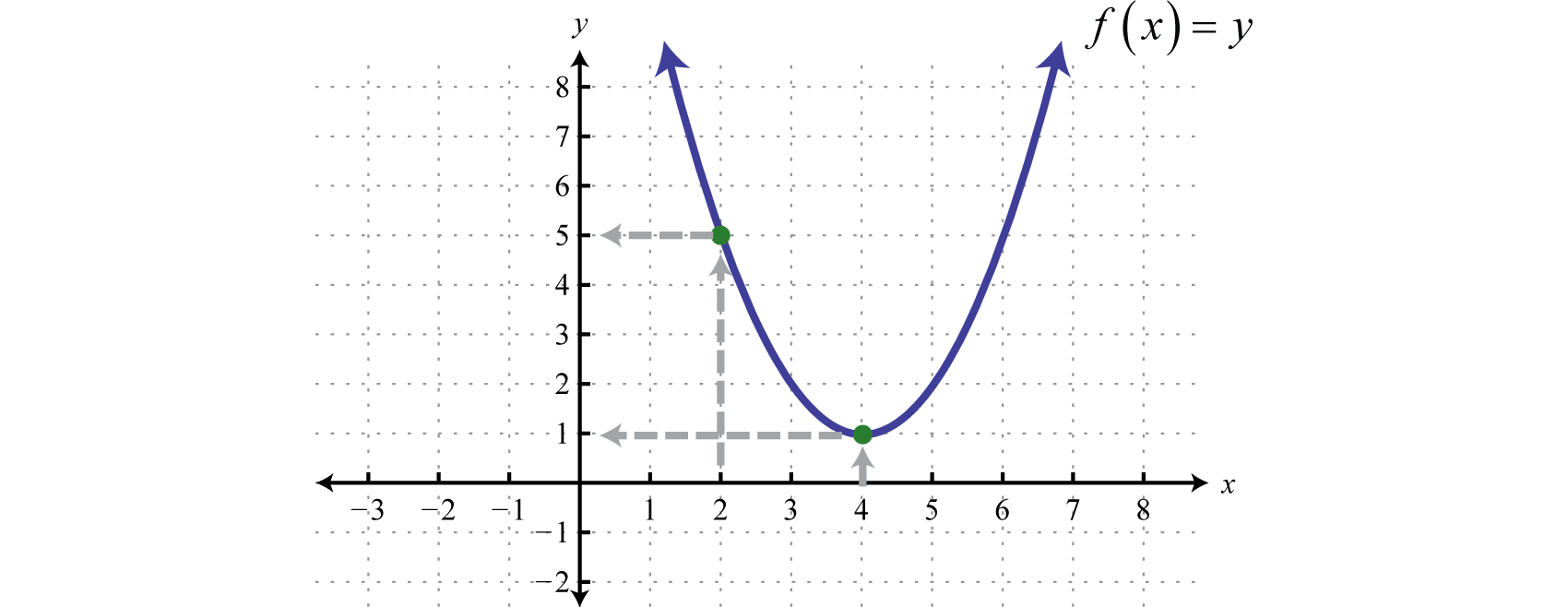Answer: $f(2)=5$; $f(4)=1$

Often we will be asked to evaluate polynomials for algebraic expressions.

### Example 2

Given $g(x)=x3−x+5$, find $g(−2u)$ and $g(x−2).$

Solution:

Replace x with the expressions given inside the parentheses.

 $g(−2u)=(−2u)3−(−2u)+5=−8u3+2u+5$ $g(x−2)=(x−2)3−(x−2)+5=(x−2)(x−2)(x−2)−(x−2)+5=(x−2)(x2−4x+4)−x+2+5=x3−4x2+4x−2x2+8x−8−x+7=x3−6x2+11x−1$

Answer: $g(−2u)=−8u2+2u+5$ and $g(x−2)=x3−6x2+11x−1$

The height of an object launched upward, ignoring the effects of air resistance, can be modeled with the following quadratic function:

$h(t)=−12gt2+v0t+s0$

With this formula, the height $h(t)$ can be calculated at any given time t after the object is launched. The letter g represents acceleration due to gravity on the surface of the Earth, which is 32 feet per second squared (or, using metric units, g = 9.8 meters per second squared). The variable $v0$, pronounced “v-naught,” or sometimes “v-zero,” represents the initial velocity of the object, and $s0$ represents the initial height from which the object was launched.

### Example 3

An object is launched from the ground at a speed of 64 feet per second. Write a function that models the height of the object and use it to calculate the objects height at 1 second and at 3.5 seconds.

Solution:

We know that the acceleration due to gravity is $g=32$ feet per second squared and we are given the initial velocity $v0=64$ feet per second. Since the object is launched from the ground, the initial height is $s0=0$ feet. Create the mathematical model by substituting these coefficients into the following formula:

$h(t)=−12gt2+v0t+s0h(t)=−12(32)t2+(64)t+0h(t)=−16t2+64t$

Use this model to calculate the height of the object at 1 second and 3.5 seconds.

$h(1)=−16(1)2+64(1)=−16+64=48h(3.5)=−16(3.5)2+64(3.5)=−196+224=28$

Answer: $h(t)=−16t2+64t$; At 1 second the object is at a height of 48 feet, and at 3.5 seconds it is at a height of 28 feet.

Try this! An object is dropped from a height of 6 meters. Write a function that models the height of the object and use it to calculate the object’s height 1 second after it is dropped.

Answer: $h(t)=−4.9t2+6$; At 1 second the object is at a height of 1.1 meters.

The notation used to indicate additionAdd functions as indicated by the notation: $(f+g)(x)=f(x)+g(x).$ and subtractionSubtract functions as indicated by the notation: $(f−g)(x)=f(x)−g(x).$ of functions follows:

$Addition of functions:(f+g)(x)=f(x)+g(x)Subtraction of functions:(f−g)(x)=f(x)−g(x)$

When using function notation, be careful to group the entire function and add or subtract accordingly.

### Example 4

Given $f(x)=x3−5x−7$ and $g(x)=3x2+7x−2$, find $(f+g)(x)$ and $(f−g)(x).$

Solution:

The notation $f+g$ indicates that we should add the given expressions.

$(f+g)(x)=f(x)+g(x)=(x3−5x−7)+(3x2+7x−2)=x3−5x−7+3x2+7x−2=x3+3x2+2x−9$

The notation $f−g$ indicates that we should subtract the given expressions. When subtracting, the parentheses become very important. Recall that we can eliminate them after applying the distributive property.

$(f−g)(x)=f(x)−g(x)=(x3−5x−7)−(3x2+7x−2)=x3−5x−7−3x2−7x+2=x3−3x2−12x−5$

Answer: $(f+g)(x)=x3+3x2+2x−9$ and $(f−g)(x)=x3−3x2−12x−5$

We may be asked to evaluate the sum or difference of two functions. We have the option to first find the sum or difference in general and then use the resulting function to evaluate for the given variable, or evaluate each first and then find the sum or difference.

### Example 5

Evaluate $(f−g)(3)$ given $f(x)=5x2−x+4$ and $g(x)=x2+2x−3.$

Solution:

First, find $(f−g)(x).$

$(f−g)(x)=f(x)−g(x)=(5x2−x+4 )−(x2+2x−3)=5x2−x+4−x2−2x+3=4x2−3x+7$

Therefore,

$(f−g)(x)=4x2−3x+7.$

Next, substitute 3 in for the variable x.

$(f−g)(3)=4(3)2−3(3)+7=36−9+7=34$

Hence $(f−g)(3)=34.$

Alternate Solution: Since $(f−g)(3)=f(3)−g(3)$, we can find $f(3)$ and $g(3)$ and then subtract the results.

 $f(x)=5x2−x+4f(3)=5(3)2−(3)+4=45−3+4=46$ $g(x)=x2+2x−3g(3)=(3)2+2(3)−3=9+6−3=12$

Therefore,

$(f−g)(3)=f(3)−g(3)=46−12=34$

Notice that we obtain the same answer.

Answer: $(f−g)(3)=34$

Note: If multiple values are to be evaluated, it is best to find the sum or difference in general first and then use it to evaluate.

Try this! Evaluate $(f+g)(−1)$ given $f(x)=x3+x−8$ and $g(x)=2x2−x+9.$

## Multiplying and Dividing Functions

The notation used to indicate multiplicationMultiply functions as indicated by the notation: $(f⋅g)(x)=f(x)⋅g(x).$ and divisionDivide functions as indicated by the notation: $(f/g)(x)=f(x)g(x)$, where $g(x)≠0.$ of functions follows:

 Multiplication of functions: $(f⋅g)(x)=f(x)⋅g(x)$ Division of functions: $(f/g)(x)=f(x)g(x), where g(x)≠0.$

### Example 6

Given $f(x)=15x4−9x3+6x2$ and $g(x)=3x2$, find $(f⋅g)(x)$ and $(f/g)(x).$

Solution:

The notation $f⋅g$ indicates that we should multiply. Apply the distributive property and simplify.

$(f⋅g)(x)=f(x)⋅g(x)=(15x4−9x3+6x2)(3x2)=15x4⋅3x2−9x3⋅3x2+6x2⋅3x2=45x6−27x5+18x4$

The notation $f/g$ indicates that we should divide. For this quotient, assume $x≠0.$

$(f/g)(x)=f(x)g(x)=15x4−9x3+6x23x2=15x43x2−9x33x2+6x23x2=5x2−3x+2$

Answer: $(f⋅g)(x)=45x6−27x5+18x4$ and $(f/g)(x)=5x2−3x+2$ where $x≠0.$

### Example 7

Given $f(x)=6x−5$ and $g(x)=3x2−2x−1$, evaluate $(f⋅g)(0)$ and $(f⋅g)(−1)$

Solution:

Begin by finding $(f⋅g)(x).$

$(f⋅g)(x)=f(x)⋅g(x)=(6x−5)(3x2−2x−1)=18x3−12x2−6x−15x2+10x+5=18x3−27x2+4x+5$

Therefore $(f⋅g)(x)=18x3−27x2+4x+5$, and we have,

 $(f⋅g)(0)=18(0)3−27(0)2+4(0)+5=5$ $(f⋅g)(−1)=18(−1)3−27(−1)2+4(−1)+5=−18−27−4+5=−44$

Answer: $(f⋅g)(0)=5$ and $(f⋅g)(−1)=−44$

Try this! Evaluate $(f⋅g)(−1)$ given $f(x)=x3+x−8$ and $g(x)=2x2−x+9.$

Here we explore the geometry of adding functions. One way to do this is to use the fact that $(f+g)(x)=f(x)+g(x).$ Add the functions together using x-values for which both $f$ and $g$ are defined.

### Example 8

Use the graphs of $f$ and $g$ to graph $f+g.$ Also, give the domain of $f+g.$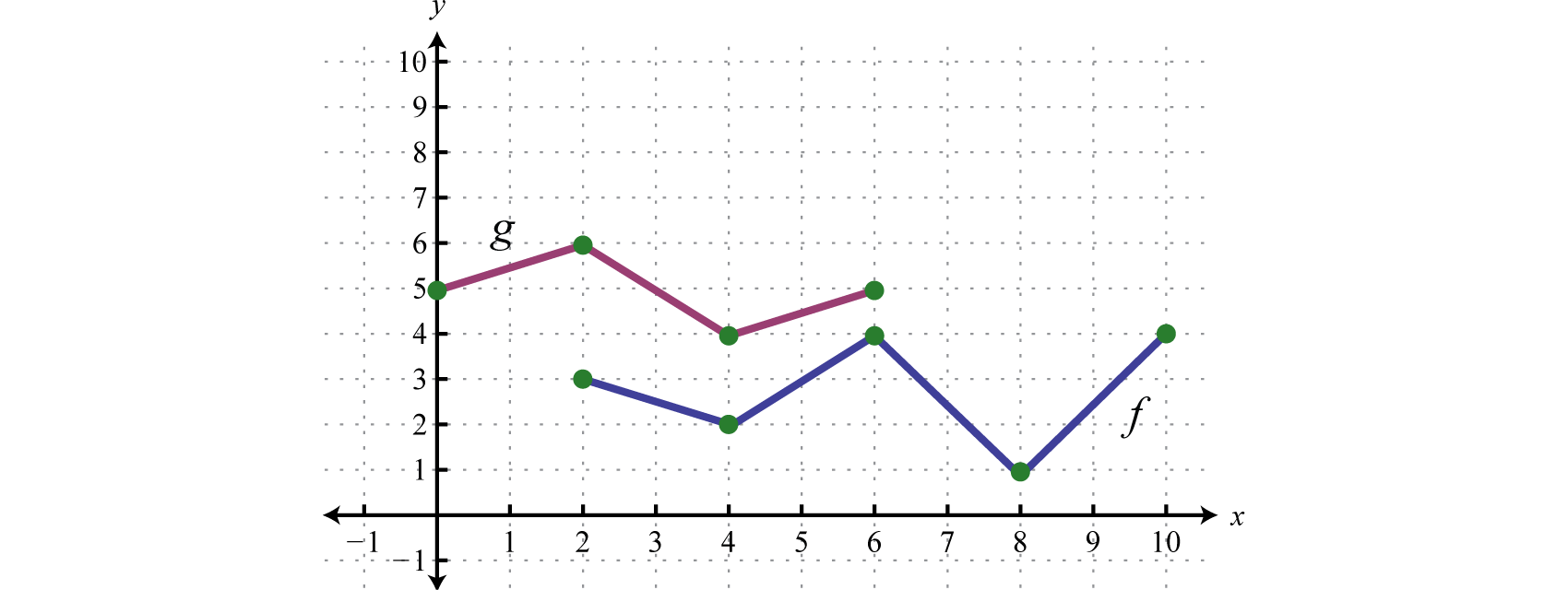Solution:

In this case, both functions are defined for x-values between 2 and 6. We will use 2, 4, and 6 as representative values in the domain of $f+g$ to sketch its graph.

$(f+g)(2)=f(2)+g(2)=3+6=9(f+g)(4)=f(4)+g(4)=2+4=6(f+g)(6)=f(6)+g(6)=4+5=9$

Sketch the graph of $f+g$ using the three ordered pair solutions $(2,9)$, $(4,6)$, and $(6,9).$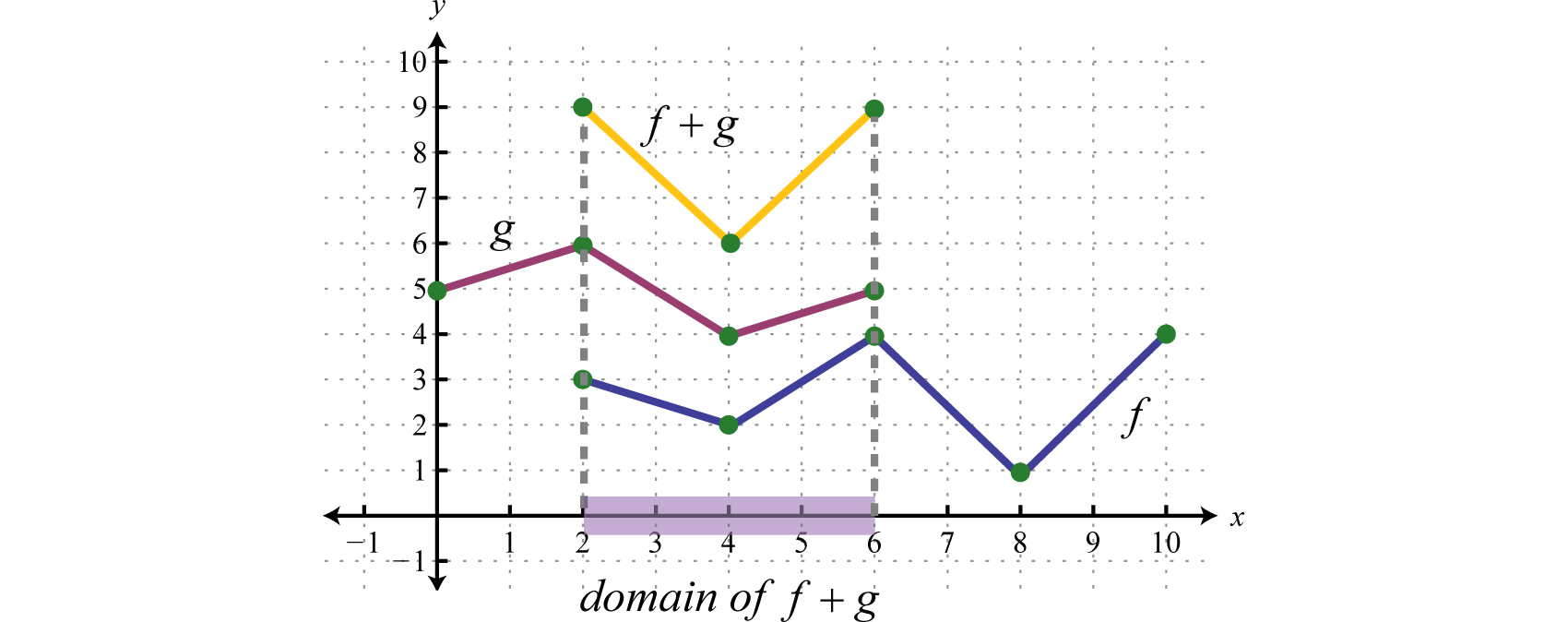Answer: $f+g$ graphed above has domain $[2,6].$

### Example 9

Use the graphs of $f$ and $g$ to graph $f+g.$ Also, give the domain of $f+g.$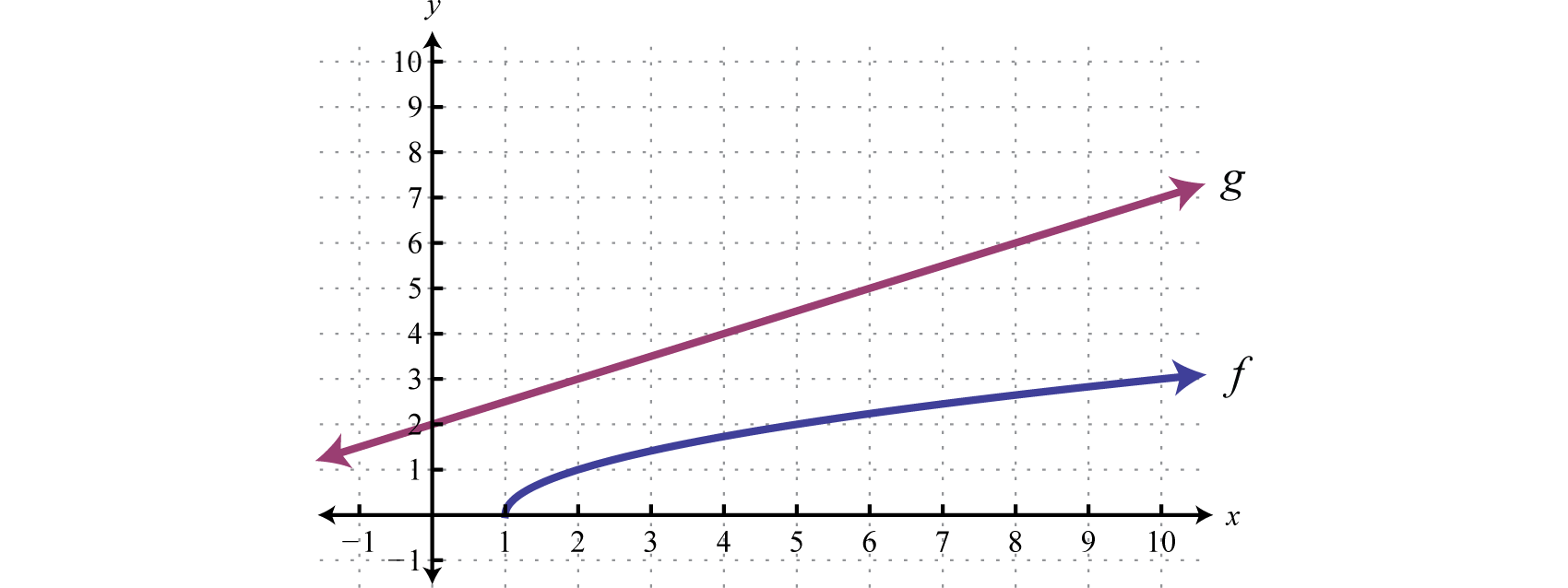Solution:

Another way to add nonnegative functions graphically is to copy the line segment formed from the x-axis to one of the functions onto the other as illustrated below.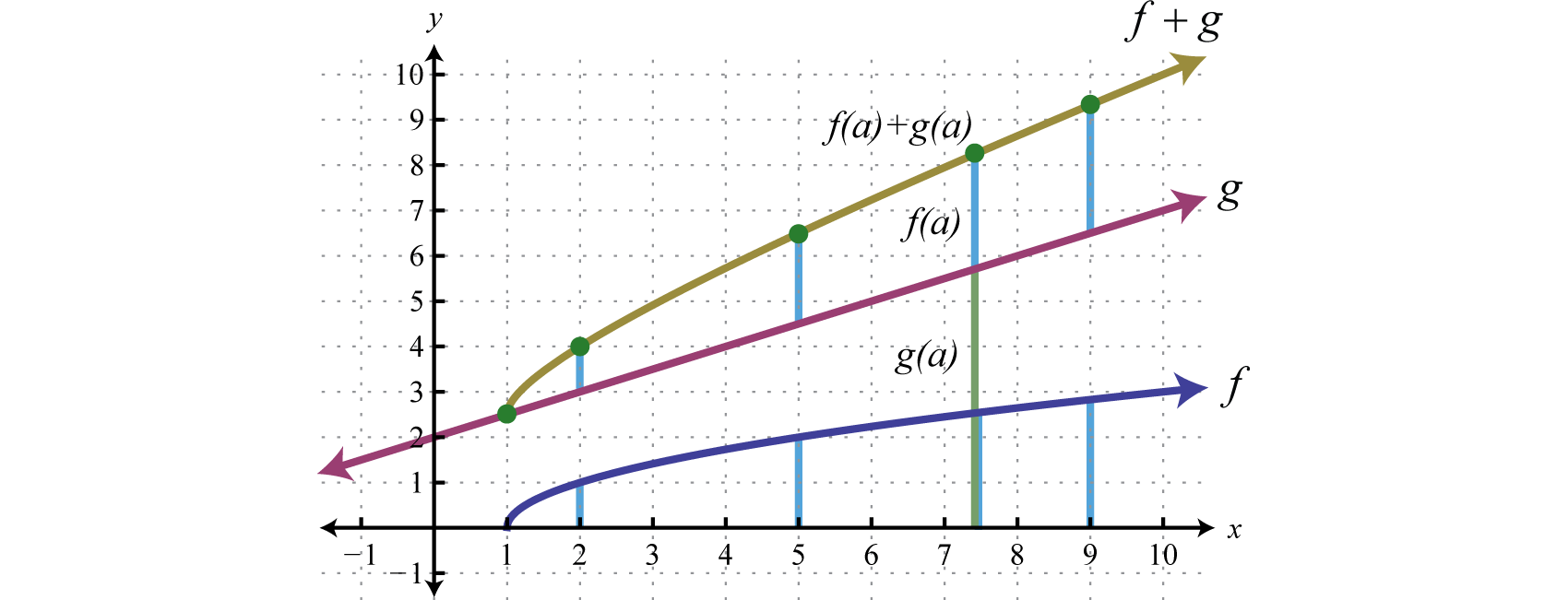The line segment from the x-axis to the function $f$ represents $f(a).$ Copy this line segment onto the other function over the same point; the endpoint represents $f(a)+g(a).$ Doing this for a number of points allows us to obtain a quick sketch of the combined graph. In this example, the domain of $f+g$ is limited to the x-values for which $f$ is defined.

Answer: Domain: $[1,∞)$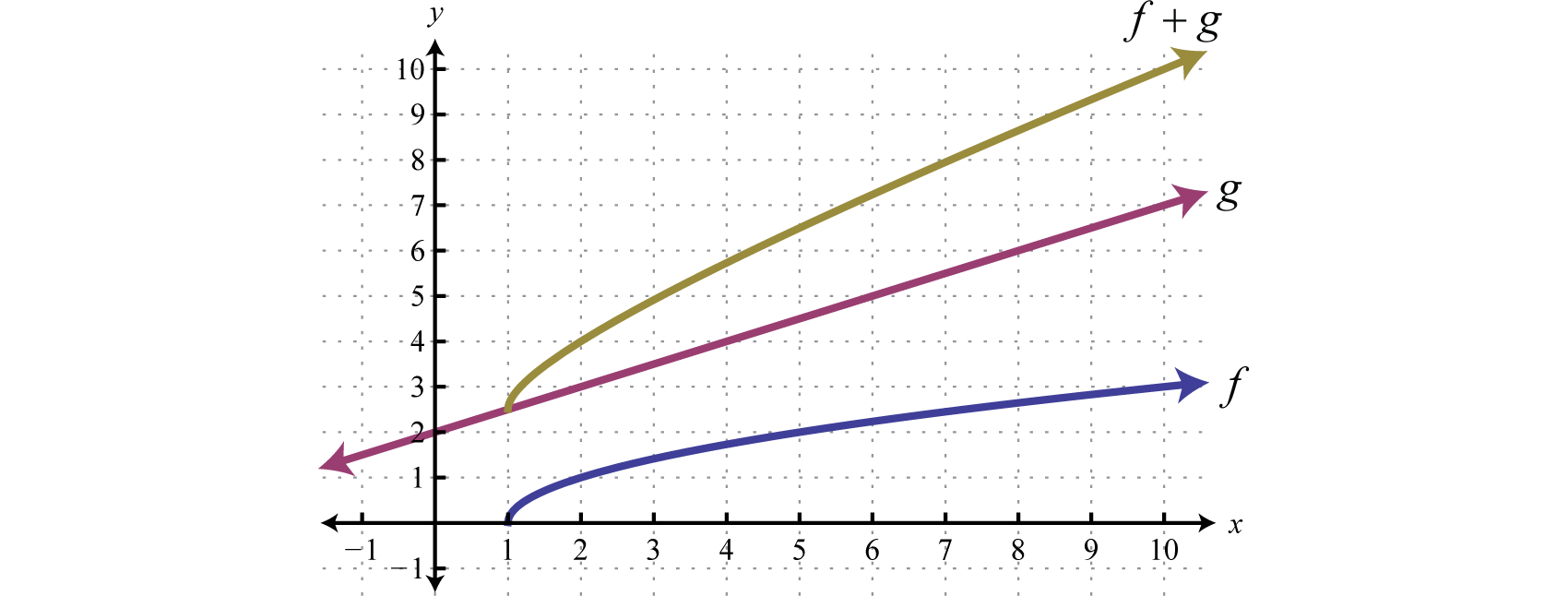In general, the domain of $f+g$ is the intersection of the domain of $f$ with the domain of $g.$ In fact, this is the case for all of the arithmetic operations with an extra consideration for division. When dividing functions, we take extra care to remove any values that make the denominator zero. This will be discussed in more detail as we progress in algebra.

### Key Takeaways

• Any polynomial with one variable is a function and can be written in the form $f(x)=anxn+an−1xn−1+…+a1x+a0.$ The degree of the polynomial is the largest exponent of all the terms.
• Use function notation to streamline the evaluating process. Substitute the value or expression inside the parentheses for each instance of the variable.
• The notation $(f+g)(x)$ indicates that we should add $f(x)+g(x).$
• The notation $(f−g)(x)$ indicates that we should subtract $f(x)−g(x).$
• The notation $(f⋅g)(x)$ indicates that we should multiply $f(x)g(x).$
• The notation $(f/g)(x)$ indicates that we should divide $f(x)g(x), where g(x)≠0.$
• The domain of the function that results from these arithmetic operations is the intersection of the domain of each function. The domain of a quotient is further restricted to values that do not evaluate to zero in the denominator.

### Part A: Polynomial Functions

Evaluate.

1. Given $f(x)=x2−10x+3$, find $f(−3)$, $f(0)$, and $f(5).$

2. Given $f(x)=2x2−x+9$, find $f(−1)$, $f(0)$, and $f(3).$

3. Given $g(x)=x3−x2+x+7$, find $g(−2)$, $g(0)$, and $g(3).$

4. Given $g(x)=x3−2x+5$, find $g(−5)$, $g(0)$, and $g(3).$

5. Given $s(t)=5t4−t2+t−3$, find $s(−1)$, $s(0)$, and $s(2).$

6. Given $p(n)=n4−10n2+9$, find $p(−3)$, $p(−1)$, and $p(2).$

7. Given $f(x)=x6−64$, find $f(−2)$, $f(−1)$, and $f(0).$

8. Given $f(x)=x6−x3+3$, find $f(−2)$, $f(−1),$ and $f(0).$

9. Given $f(x)=x2−2x−1$, find $f(2t)$ and $f(2t−1).$

10. Given $f(x)=x2−2x+4$, find $f(−3t)$ and $f(2−3t).$

11. Given $g(x)=2x2+3x−1$, find $g(−5a)$ and $g(5−2x).$

12. Given $g(x)=3x2−5x+4$, find $g(−4u)$ and $g(3x−1).$

13. Given $f(x)=x3−1$, find $f(2a)$ and $f(x−2).$

14. Given $f(x)=x3−x+1$, find $f(−3x)$ and $f(2x+1).$

15. Given $g(x)=x3+x2−1$, find $g(x2)$ and $g(x−4).$

16. Given $g(x)=2x3−x+1$, find $g(−2x3)$ and $g(3x−1).$

Given the function calculate $f(x+h).$

1. $f(x)=5x−3$

2. $f(x)=x2−1$

3. $f(x)=x3−8$

4. $f(x)=x4$

Given the graph of the polynomial function $f$ find the function values.

1. Find $f(0)$, $f(1)$, and $f(2).$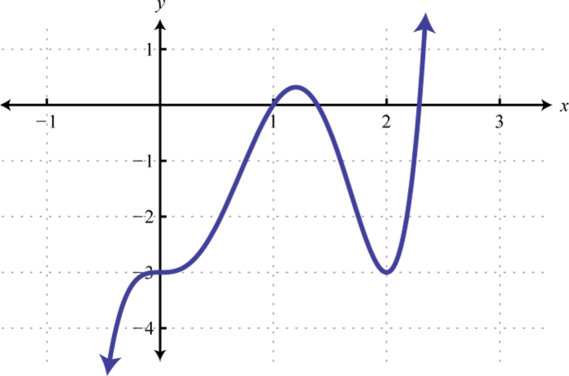2. Find $f(−1)$, $f(0)$, and $f(1).$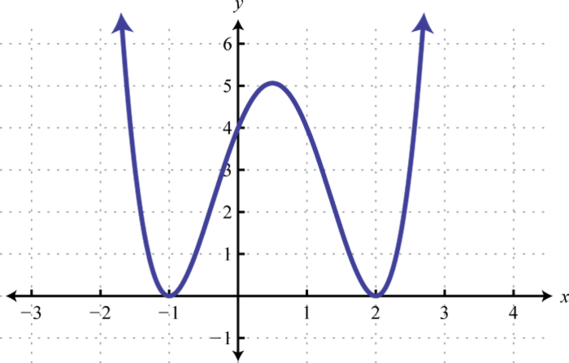3. Find $f(−2)$, $f(−1)$, and $f(0).$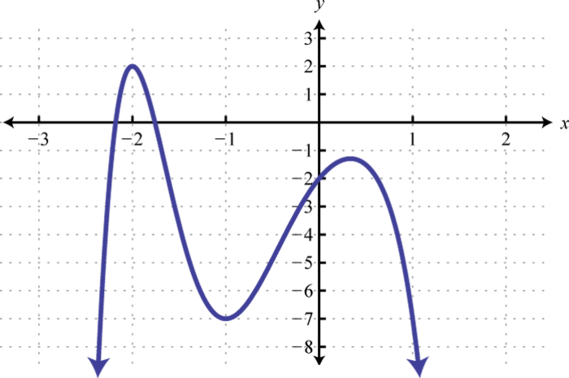4. Find $f(−3)$, $f(−2)$, and $f(0).$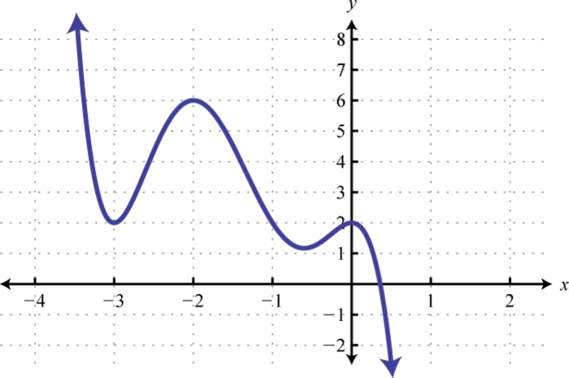5. A projectile is launched upward from the ground at a speed of 48 feet per second. Write a function that models the height of the projectile and use it to calculate the height every 1/2 second after launch. Sketch a graph that shows the height of the projectile with respect to time.

6. An object is tossed upward from a 48-foot platform at a speed of 32 feet per second. Write a function that models the height of the object and use it to calculate the height every 1/2 second after the object is tossed. Sketch a graph that shows the height of the object with respect to time.

7. An object is dropped from a 128-foot bridge. Write a function that models the height of the object, and use it to calculate the height at 1 second and 2 seconds after it has been dropped.

8. An object is dropped from a 500-foot building. Write a function that models the height of the object, and use it to calculate the distance the object falls in the 1st second, 2nd second, and the 3rd second.

9. A bullet is fired straight up into the air at 320 meters per second. Ignoring the effects of air friction, write a function that models the height of the bullet, and use it to calculate the bullet’s height 1 minute after it was fired into the air.

10. A book is dropped from a height of 10 meters. Write a function that gives the height of the book, and use it to determine how far it will fall in 1¼ seconds.

### Part B: Adding and Subtracting Functions

Given functions $f$ and $g$, find $(f+g)$ and $(f−g).$

1. $f(x)=5x−3$, $g(x)=4x−1$

2. $f(x)=3x+2$, $g(x)=7x−5$

3. $f(x)=2−3x$, $g(x)=1−x$

4. $f(x)=8x−5$, $g(x)=−7x+4$

5. $f(x)=x2−3x+2$, $g(x)=x2+4x−7$

6. $f(x)=2x2+x−3$, $g(x)=x2−x+4$

7. $f(x)=x2+5x−3$, $g(x)=6x+11$

8. $f(x)=9x+5$, $g(x)=2x2−5x+4$

9. $f(x)=9x2−1$, $g(x)=x2+5x$

10. $f(x)=10x2$, $g(x)=5x2−8$

11. $f(x)=8x3+x−4$, $g(x)=4x3+x2−1$

12. $f(x)=x3−x2+x+1$, $g(x)=x3−x2−x−1$

Given $f(x)=x3+2x2−8$ and $g(x)=2x2−3x+5$, evaluate the following.

1. $(f+g)(−2)$

2. $(f+g)(3)$

3. $(f−g)(−2)$

4. $(f−g)(3)$

5. $(g−f)(−2)$

6. $(g−f)(3)$

7. $(f+f)(1)$

8. $(g+g)(−1)$

Given the graphs of $f$ and $g$, evaluate the following.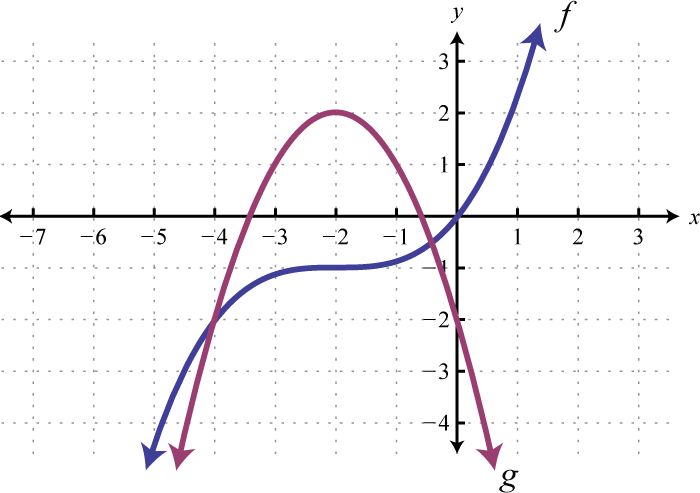1. $(f+g)(−4)$

2. $(f−g)(−4)$

3. $(f+g)(−2)$

4. $(f−g)(−2)$

5. $(f+g)(0)$

6. $(f−g)(0)$

### Part C: Multiplying and Dividing Functions

Given $f$ and $g$, find $f⋅g.$

1. $f(x)=5x$, $g(x)=x−3$

2. $f(x)=x−4$, $g(x)=6x$

3. $f(x)=2x−3$, $g(x)=3x+4$

4. $f(x)=5x−1$, $g(x)=2x+1$

5. $f(x)=3x+4$, $g(x)=3x−4$

6. $f(x)=x+5$, $g(x)=x−5$

7. $f(x)=x−2$, $g(x)=x2−3x+2$

8. $f(x)=2x−3$, $g(x)=x2+2x−1$

9. $f(x)=2x2$, $g(x)=x2−7x+5$

10. $f(x)=5x3$, $g(x)=x2−3x−1$

11. $f(x)=x2−3x−2$, $g(x)=2x2−x+3$

12. $f(x)=x2+x−1$, $g(x)=x2−x+1$

Given $f$ and $g$, find $f/g.$ (Assume all expressions in the denominator are nonzero.)

1. $f(x)=36x3−16x2−8x$, $g(x)=4x$

2. $f(x)=2x3−6x2+10x$, $g(x)=2x$

3. $f(x)=20x7−15x5+5x3$, $g(x)=5x3$

4. $f(x)=9x6+12x4−3x2$, $g(x)=3x2$

5. $f(x)=x3+4x2+3x−2$, $g(x)=x+2$

6. $f(x)=x3−x2−10x+12$, $g(x)=x−3$

7. $f(x)=6x3−13x2+36x−45$, $g(x)=2x−3$

8. $f(x)=6x3−11x2+15x−4$, $g(x)=3x−1$

9. $f(x)=3x3−13x2−x+8$, $g(x)=3x+2$

10. $f(x)=5x3−16x2+13x−6$, $g(x)=5x−1$

Given $f(x)=25x4+10x3−5x2$ and $g(x)=5x2$ evaluate the following.

1. $(f⋅g)(−1)$

2. $(f⋅g)(1)$

3. $(f/g)(−2)$

4. $(f/g)(−3)$

5. $(g⋅f)(0)$

6. $(g/f)(1)$

7. $(g⋅g)(−1)$

8. $(f⋅f)(−1)$

Given the graphs of $f$ and $g$ evaluate the following.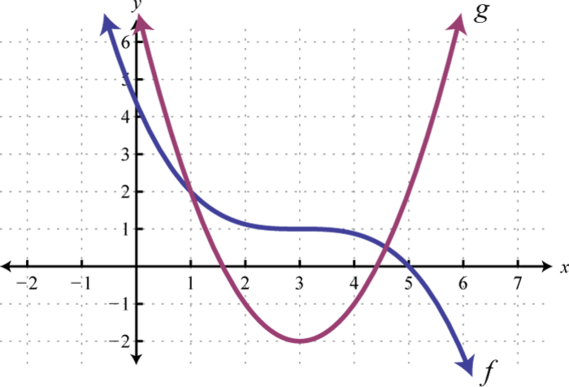1. $(f⋅g)(3)$

2. $(f⋅g)(5)$

3. $(f/g)(5)$

4. $(f/g)(3)$

5. $(f⋅g)(1)$

6. $(f/g)(1)$

Given $f(x)=5x3−15x2+10x$, $g(x)=x2−x+3$, and $h(x)=−5x$, find the following. (Assume all expressions in the denominator are nonzero.)

1. $(f−g)(x)$

2. $(g−f)(x)$

3. $(g⋅h)(x)$

4. $(f/h)(x)$

5. $(h+g)(x)$

6. $(h⋅f)(x)$

7. $(g/h)(2)$

8. $(g−h)(−3)$

9. The revenue in dollars from selling MP3 players is given by the function $R(n)=125n−0.15n2$, where n represents the number of units sold $(0≤n<833)$. The cost in dollars of producing the MP3 players is given by the formula $C(n)=1200+42n$ where n represents the number of units produced. Write a function that models the profit of producing and selling n MP3 players. Use the function to determine the profit generated from producing and selling 225 MP3 players. Recall that profit equals revenues less costs.

10. The inner radius of a washer is $12$ that of the outer radius.### Part D: Adding Functions Geometrically

Use the graphs of $f$ and $g$ to graph $f+g.$ Also, give the domain of $f+g.$

1.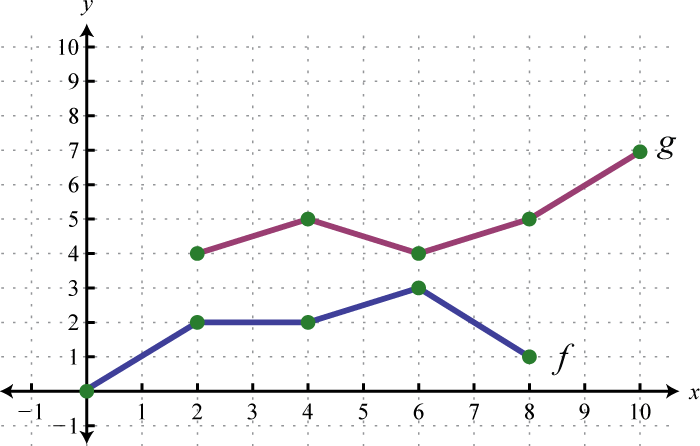2.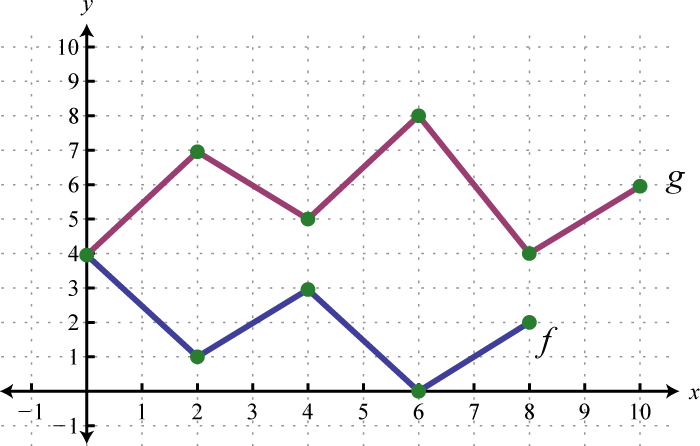3.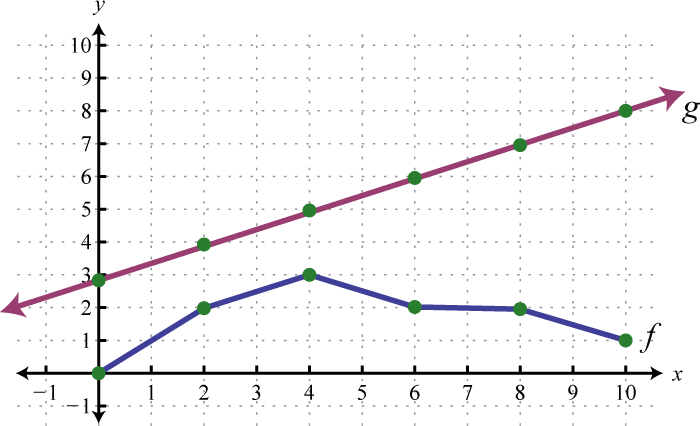4.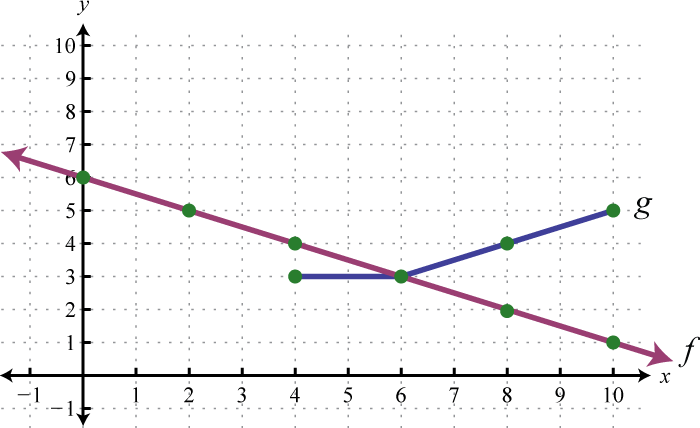5.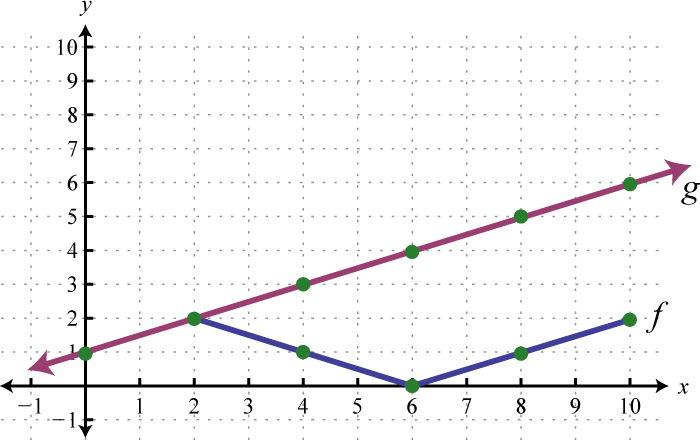6.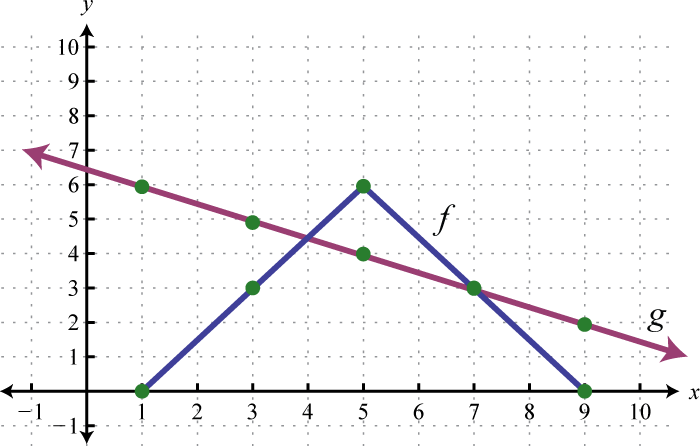7.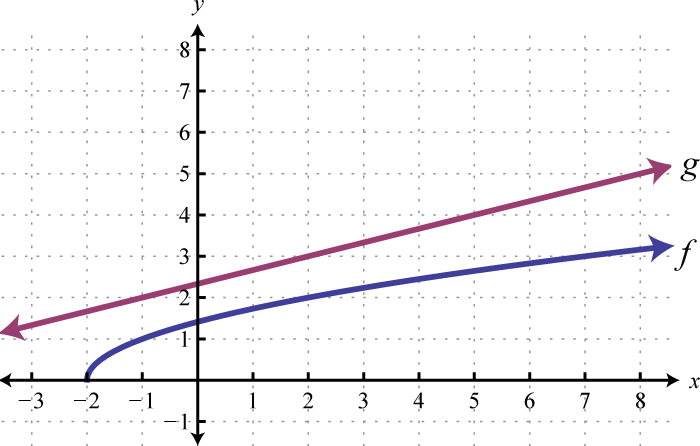8.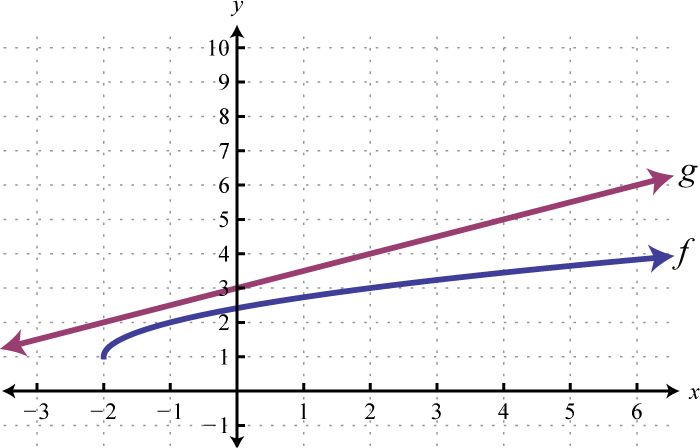9.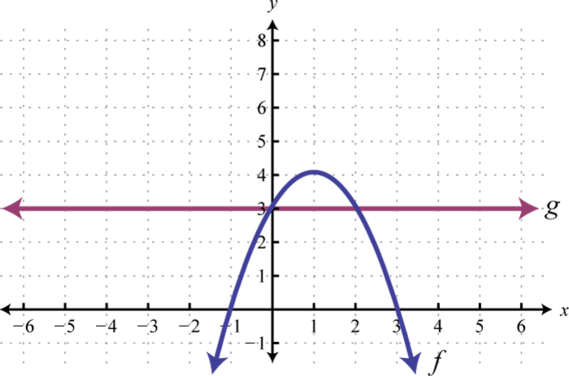10.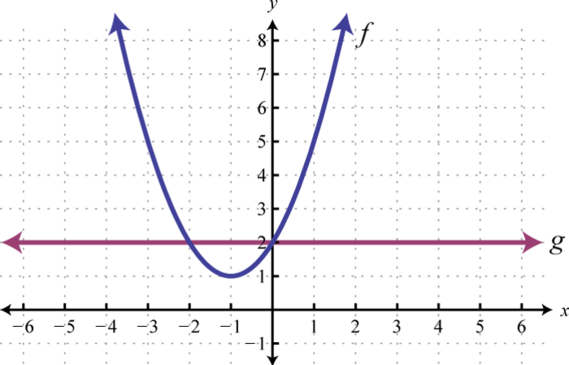### Part E: Discussion Board

1. Which arithmetic operations on functions are commutative? Explain.

2. Explore ways we can add functions graphically if they happen to be negative.

1. $f(−3)=42$; $f(0)=3$; $f(5)=−22$

2. $g(−2)=−7$; $g(0)=7$; $g(3)=28$

3. $s(−1)=0$; $s(0)=−3$; $s(2)=75$

4. $f(−2)=0$; $f(−1)=−63$; $f(0)=−64$

5. $f(2t)=4t2−4t−1$; $f(2t−1)=4t2−8t+2$

6. $g(−5a)=50a2−15a−1$; $g(5−2x)=8x2−46x+64$

7. $f(2a)=8a3−1$; $f(x−2)=x3−6x2+12x−9$

8. $g(x2)=x6+x4−1$; $g(x−4)=x3−11x2+40x−49$

9. $f(x+h)=5x+5h−3$

10. $f(x+h)=x3+3hx2+3h2x+h3−8$

11. $f(0)=−3$; $f(1)=0$; $f(2)=−3$

12. $f(−2)=2$; $f(−1)=−7$; $f(0)=−2$

13. $h(t)=−16t2+48t$;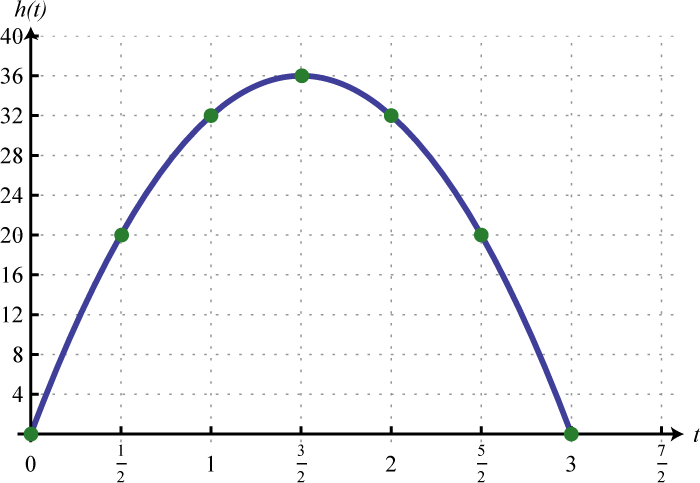14. $h(t)=−16t2+128$; At 1 second the object’s height is 112 feet and at 2 seconds its height is 64 feet.

15. $h(t)=−4.9t2+320t$; 1,560 meters

1. $(f+g)(x)=9x−4$; $(f−g)(x)=x−2$

2. $(f+g)(x)=−4x+3$; $(f−g)(x)=−2x+1$

3. $(f+g)(x)=2x2+x−5$; $(f−g)(x)=−7x+9$

4. $(f+g)(x)=x2+11x+8$; $(f−g)(x)=x2−x−14$

5. $(f+g)(x)=10x2+5x−1$; $(f−g)(x)=8x2−5x−1$

6. $(f+g)(x)=12x3+x2+x−5$; $(f−g)(x)=4x3−x2+x−3$

7. 11

8. −27

9. 27

10. −10

11. −4

12. 1

13. −2

1. $(f⋅g)(x)=5x2−15x$

2. $(f⋅g)(x)=6x2−x−12$

3. $(f⋅g)(x)=9x2−16$

4. $(f⋅g)(x)=x3−5x2+8x−4$

5. $(f⋅g)(x)=2x4−14x3+10x2$

6. $(f⋅g)(x)=2x4−7x3+2x2−7x−6$

7. $(f/g)(x)=9x2−4x−2$

8. $(f/g)(x)=4x4−3x2+1$

9. $(f/g)(x)=x2+2x−1$

10. $(f/g)(x)=3x2−2x+15$

11. $(f/g)(x)=x2−5x+3+23x+2$

12. 50

13. 15

14. 0

15. 25

16. −2

17. 0

18. 4

19. $(f−g)(x)=5x3−16x2+11x−3$

20. $(g⋅h)(x)=−5x3+5x2−15x$

21. $(h+g)(x)=x2−6x+3$

22. $(g/h)(2)=−12$

23. $P(n)=−0.15n2+83n−1200$; \$9,881.25

1.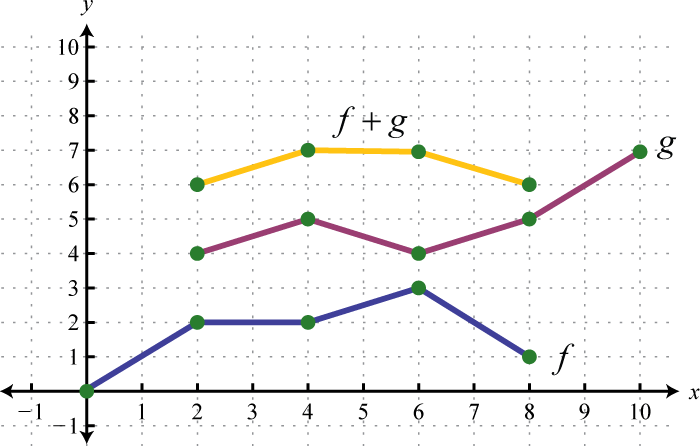$[2,8]$
2.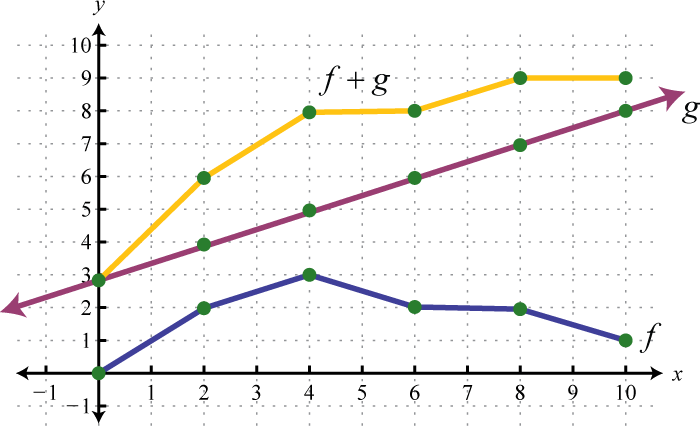$[0,10]$
3.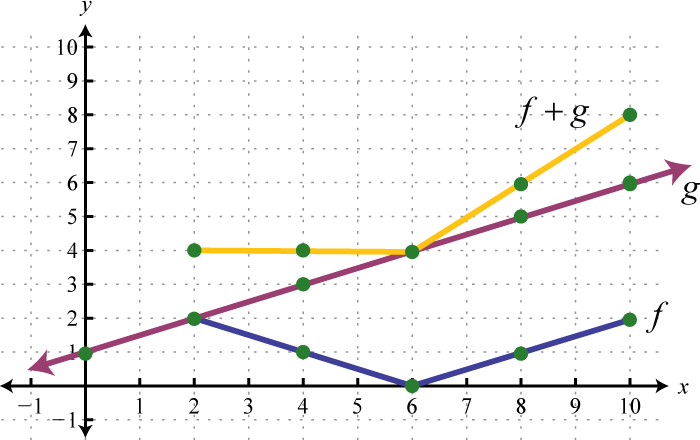$[2,10]$
4.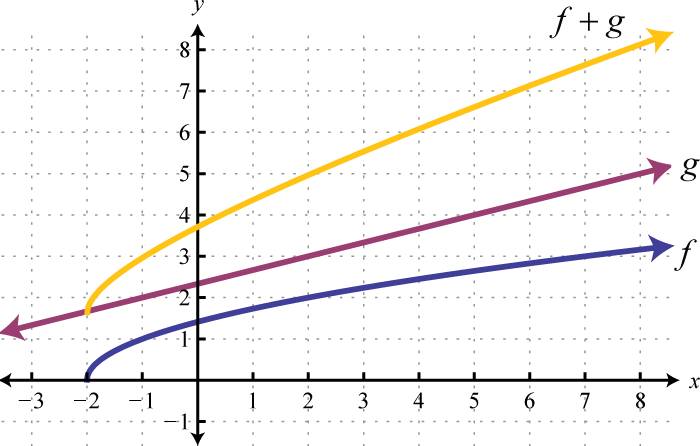$[−2,∞)$
5.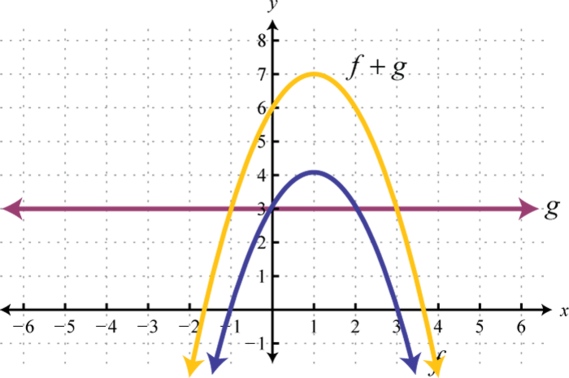$(−∞,∞)$

## 4.2 Factoring Polynomials

### Learning Objectives

1. Determine the greatest common factor (GCF) of monomials.
2. Factor out the GCF of a polynomial.
3. Factor a four-term polynomial by grouping.
4. Factor special binomials.

## Determining the GCF of Monomials

The process of writing a number or expression as a product is called factoringThe process of writing a number or expression as a product.. If we write the monomial $8x7=2x5⋅4x2$, we say that the product $2x5⋅4x2$ is a factorizationAny combination of factors, multiplied together, resulting in the product. of $8x7$ and that $2x5$ and $4x2$ are factorsAny of the numbers or expressions that form a product.. Typically, there are many ways to factor a monomial. Some factorizations of $8x7$ follow:

$8x7=2x5⋅4x28x7=8x6⋅x8x7=2x⋅2x2⋅2x4} Factorizations of 8x7$

Given two or more monomials, it will be useful to find the greatest common monomial factor (GCF)The product of the common variable factors and the GCF of the coefficients. of each. The GCF of the monomials is the product of the common variable factors and the GCF of the coefficients.

### Example 1

Find the GCF of $25x7y2z$ and $15x3y4z2.$

Solution:

Begin by finding the GCF of the coefficients. In this case, $25=5⋅5$ and $15=3⋅5.$ It should be clear that

$GCF(25,15)=5$

Next determine the common variable factors with the smallest exponents.

$25x7y2z and 15x3y4z2$

The common variable factors are $x3$, $y2$, and $z.$ Therefore, given the two monomials,

$GCF=5x3y2z$

Answer: $5x3y2z$

It is worth pointing out that the GCF divides both expressions evenly.

$25x7y2z 5x3y2z=5x4and15x3y4z25x3y2z=3y2z$

Furthermore, we can write the following:

$25x7y2z =5x3y2z⋅5x4and15x3y4z2=5x3y2z⋅3y2z$

The factors $5x4$ and $3y2z$ share no common monomial factors other than 1; they are relatively primeExpressions that share no common factors other than 1..

### Example 2

Determine the GCF of the following three expressions: $12a5b2(a+b)5$, $60a4b3c (a+b)3$, and $24a2b7c3(a+b)2.$

Solution:

Begin by finding the GCF of the coefficients. To do this, determine the prime factorization of each and then multiply the common factors with the smallest exponents.

$12=22⋅360=22⋅3⋅524=23⋅3$

Therefore, the GCF of the coefficients of the three monomials is

$GCF(12,60,24)=22⋅3=12$

Next, determine the common factors of the variables.

$12a5b2(a+b)5and60a4b3c (a+b)3and24a2b7c3(a+b)2$

The variable factors in common are $a2$, $b2$, and $(a+b)2.$ Therefore,

$GCF=12⋅a2⋅b2⋅(a+b)2$

Note that the variable c is not common to all three expressions and thus is not included in the GCF.

Answer: $12a2b2(a+b)2$

## Factoring out the GCF

The application of the distributive property is the key to multiplying polynomials. For example,

$6xy2(2xy+1)=6xy2⋅2xy+6xy2⋅1Multiplying=12x2y3 + 6xy2$

The process of factoring a polynomial involves applying the distributive property in reverse to write each polynomial as a product of polynomial factors.

$a(b+c)=ab+acMultiplyingab+ac=a(b+c)Factoring$

Consider factoring the result of the opening example:

$12x2y3 + 6xy2=6xy2⋅2xy + 6xy2⋅1Factoring=6xy2( ? )=6xy2(2xy+1)$

We see that the distributive property allows us to write the polynomial $12x2y3 + 6xy2$ as a product of the two factors $6xy2$ and $(2xy+1).$ Note that in this case, $6x2y$ is the GCF of the terms of the polynomial.

$GCF(12x2y3, 6xy2)=6xy2$

Factoring out the greatest common factor (GCF)The process of rewriting a polynomial as a product using the GCF of all of its terms. of a polynomial involves rewriting it as a product where a factor is the GCF of all of its terms.

$8x3+4x2−16x=4x(2x2+x−4)9ab2−18a2b−3ab=3ab(3b−6a−1)}Factoring out the GCF$

To factor out the GCF of a polynomial, we first determine the GCF of all of its terms. Then we can divide each term of the polynomial by this factor as a means to determine the remaining factor after applying the distributive property in reverse.

### Example 3

Factor out the GCF: $18x7−30x5+6x3.$

Solution:

In this case, the GCF(18, 30, 6) = 6, and the common variable factor with the smallest exponent is $x3.$ The GCF of the polynomial is $6x3.$

$18x7−30x5+6x3=6x3(?)$

The missing factor can be found by dividing each term of the original expression by the GCF.

$18x76x3=3x4 −30x56x3=−5x2 +6x36x3=+1$

Apply the distributive property (in reverse) using the terms found in the previous step.

$18x7−30x5+6x3=6x3(3x4−5x2+1)$

If the GCF is the same as one of the terms, then, after the GCF is factored out, a constant term 1 will remain. The importance of remembering the constant term becomes clear when performing the check using the distributive property.

$6x3(3x4−5x2+1)=6x3⋅3x4−6x3⋅5x2+6x3⋅1=18x7−30x5+6x3✓$

Answer: $6x3(3x4−5x2+1)$

### Example 4

Factor out the GCF: $27x5y5z+54x5yz−63x3y4.$

Solution:

The GCF of the terms is $9x3y.$ The last term does not have a variable factor of z, and thus z cannot be a part of the greatest common factor. If we divide each term by $9x3y$, we obtain

$27x5y5z9x3y=3x2y4z 54x5yz9x3y=6x2z −63x3y49x3y=−7y3$

and can write

$27x5y5z+54x5yz−63x3y4=9x3y(?)=9x3y (3x2y4z+6x2z−7y3)$

Answer: $9x3y (3x2y4z+6x2z−7y3)$

Try this! Factor out the GCF: $12x3y4−6x2y3−3xy2$

Answer: $3xy2(4x2y2−2xy−1)$

Of course, not every polynomial with integer coefficients can be factored as a product of polynomials with integer coefficients other than 1 and itself. If this is the case, then we say that it is a prime polynomialA polynomial with integer coefficients that cannot be factored as a product of polynomials with integer coefficients other than 1 and itself.. For example, a linear factor such as $10x−9$ is prime. However, it can be factored as follows:

$10x−9=x(10−9x)or10x−9=5(2x−95)$

If an x is factored out, the resulting factor is not a polynomial. If any constant is factored out, the resulting polynomial factor will not have integer coefficients. Furthermore, some linear factors are not prime. For example,

$5x−10=5(x−2)$

In general, any linear factor of the form $ax+b$, where a and b are relatively prime integers, is prime.

## Factoring by Grouping

In this section, we outline a technique for factoring polynomials with four terms. First, review a preliminary example where the terms have a common binomial factor.

### Example 5

Factor: $7x(3x−2)−(3x−2).$

Solution:

Begin by rewriting the second term $−(3x−2)$ as $−1(3x−2).$ Next, consider $(3x−2)$ as a common binomial factor and factor it out as follows:

$7x(3x−2)−(3x−2)=7x(3x−2)−1(3x−2)=(3x−2)( ? )=(3x−2)(7x−1)$

Answer: $(3x−2)(7x−1)$

Factoring by groupingA technique for factoring polynomials with four terms. is a technique that enables us to factor polynomials with four terms into a product of binomials. This involves an intermediate step where a common binomial factor will be factored out. For example, we wish to factor

$3x3−12x2+2x−8$

Begin by grouping the first two terms and the last two terms. Then factor out the GCF of each grouping:In this form, the polynomial is a binomial with a common binomial factor, $(x−4).$

$=(x−4)( ? )=(x−4)(3x2+2)$

Therefore,

$3x3−12x2+2x−8=(x−4)(3x2+2)$

We can check by multiplying.

$(x−4)(3x2+2)=3x3+2x−12x2−8=3x3−12x2+2x−8 ✓$

### Example 6

Factor by grouping: $24a4−18a3−20a+15.$

Solution:

The GCF for the first group is $6a3.$ We have to choose 5 or −5 to factor out of the second group.Factoring out +5 does not result in a common binomial factor. If we choose to factor out −5, then we obtain a common binomial factor and can proceed. Note that when factoring out a negative number, we change the signs of the factored terms.Answer: $(4a−3)(6a3−5).$ Check by multiplying; this is left to the reader as an exercise.

Sometimes we must first rearrange the terms in order to obtain a common factor.

### Example 7

Factor: $ab−2a2b+a3−2b2.$

Solution:

Simply factoring the GCF out of the first group and last group does not yield a common binomial factor.We must rearrange the terms, searching for a grouping that produces a common factor. In this example, we have a workable grouping if we switch the terms $a3$ and $ab.$Answer: $(a−2b)(a2+b)$

Try this! Factor: $x3−x2y−xy+y2.$

Answer: $(x−y)(x2−y)$

Not all factorable four-term polynomials can be factored with this technique. For example,

$3x3+5x2−x+2$

This four-term polynomial cannot be grouped in any way to produce a common binomial factor. Despite this, the polynomial is not prime and can be written as a product of polynomials. It can be factored as follows:

$3x3+5x2−x+2=(x+2)(3x2−x+1)$

Factoring such polynomials is something that we will learn to do as we move further along in our study of algebra. For now, we will limit our attempt to factor four-term polynomials to using the factor by grouping technique.

## Factoring Special Binomials

A binomial is a polynomial with two terms. We begin with the special binomial called difference of squares$a2−b2=(a+b)(a−b),$ where a and b represent algebraic expressions.:

$a2−b2=(a+b)(a−b)$

To verify the above formula, multiply.

$(a+b)(a−b)=a2−ab+ba−b2=a2−ab+ab−b2=a2−b2$

We use this formula to factor certain special binomials.

### Example 8

Factor: $x2−9y2.$

Solution:

Identify the binomial as difference of squares and determine the square factors of each term.Here we can write

$x2−9y2=( x )2−( 3y )2$

Substitute into the difference of squares formula where $a=x$ and $b=3y.$

$a2−b2=(a+b)(a−b)↓↓↓↓x2−9y2=(x+3y)(x−3y)$

Multiply to check.

$(x+3y)(x−3y)=x2−3xy+3yx−9y2=x2−3xy+3xy−9y2=x2−9y2 ✓$

Answer: $(x+3y)(x−3y)$

### Example 9

Factor: $x2−(2x−1)2.$

Solution:

First, identify this expression as a difference of squares.

$x2−(2x−1)2=( x )2−( 2x−1 )2$

Use $a=x$ and $b=2x−1$ in the formula for a difference of squares and then simplify.

$a2−b2=(a+b)(a−b)$

$x2−(2x−1)2=[ x+(2x−1)][x−(2x−1)]=( x+2x−1)(x−2x+1)=(3x−1)(−x+1)$

Answer: $(3x−1)(−x+1)$

Given any real number b, a polynomial of the form $x2+b2$ is prime. Furthermore, the sum of squares$a2+b2,$ where a and b represent algebraic expressions. This does not have a general factored equivalent. $a2+b2$ does not have a general factored equivalent. Care should be taken not to confuse this with a perfect square trinomial.

$(a+b)2=(a+b)(a+b)=a2+ab+ba+b2=a2+2ab+b2$

Therefore,

$(a+b)2≠a2+b2$

For example, the sum of squares binomial $x2+9$ is prime. Two other special binomials of interest are the sum$a3+b3=(a+b)(a2−ab+b2)$, where a and b represent algebraic expressions. and difference of cubes$a3−b3=(a−b)(a2+ab+b2)$, where a and b represent algebraic expressions.:

$a3+b3=(a+b)(a2−ab+b2)a3−b3=(a−b)(a2+ab+b2)$

We can verify these formulas by multiplying.

$(a+b)(a2−ab+b2)=a3−a2b+ab2+a2b−ab2+b3=a3+b3 ✓$

$(a−b)(a2+ab+b2)=a3+a2b+ab2−a2b−ab2−b3=a3−b3 ✓$

The process for factoring sums and differences of cubes is very similar to that of differences of squares. We first identify a and b and then substitute into the appropriate formula. The separate formulas for the sum and difference of cubes allow us to always choose a and b to be positive.

### Example 10

Factor: $x3−8y3.$

Solution:

First, identify this binomial as a difference of cubes.Next, identify what is being cubed.

$x3−8y3=(x)3−(2y)3$

In this case, $a=x$ and $b=2y.$ Substitute into the difference of cubes formula.

$a3+b3=(a−b)(a2 + a ⋅ b+b2)↓↓↓↓ ↓↓x3−8y3=(x−2y)((x)2+x⋅2y+(2y)2)=(x−2y)(x2+2xy+4y2)$

We can check this factorization by multiplying.

$(x−2y)(x2+2xy+4y2)=x3+2x2y+4xy2−2x2y−4xy2−8y3=x3 + 2x2y + 4xy2−2x2y−4xy2−8y3=x3−8y3 ✓$

Answer: $(x−2y)(x2+2xy+4y2)$

It may be the case that the terms of the binomial have a common factor. If so, it will be difficult to identify it as a special binomial until we first factor out the GCF.

### Example 11

Factor: $81x4y+3xy4.$

Solution:

The terms are not perfect squares or perfect cubes. However, notice that they do have a common factor. First, factor out the GCF, $3xy.$

$81x4y+3xy4=3xy(27x3+y3)$

The resulting binomial factor is a sum of cubes with $a=3x$ and $b=y.$

$81x4y+3xy4=3xy(27x3+y3)=3xy(3x+y)(9x2−3xy+y2)$

Answer: $3xy(3x+y)(9x2−3xy+y2)$

When the degree of the special binomial is greater than two, we may need to apply the formulas multiple times to obtain a complete factorization. A polynomial is completely factoredA polynomial that is prime or written as a product of prime polynomials. when it is prime or is written as a product of prime polynomials.

### Example 12

Factor completely: $x4−81y4.$

Solution:

First, identify what is being squared.

$x4−81y4=( )2−( )2$

To do this, recall the power rule for exponents, $(xm)n=xmn.$ When exponents are raised to a power, multiply them. With this in mind, we find

$x4−81y4=( x2 )2−( 9y2 )2$

Therefore, $a=x2$ and $b=9y2.$ Substitute into the formula for difference of squares.

$x4−81y4=(x2+9y2)(x2−9y2)$

At this point, notice that the factor $(x2−9y2)$ is itself a difference of two squares and thus can be further factored using $a=x2$ and $b=3y.$ The factor $(x2+9y2)$ is prime and cannot be factored using real numbers.

$x4−81y4=(x2+9y2)(x2−9y2)=(x2+9y2)(x+3y)(x−3y)$

Answer: $(x2+9y2)(x+3y)(x−3y)$

When factoring, always look for resulting factors to factor further.

### Example 13

Factor completely: $64x6−y6.$

Solution:

This binomial is both a difference of squares and difference of cubes.

$64x6−y6=( 4x2 )3−( y2 )3 Difference of cubes64x6−y6=( 8x3 )2−( y3 )2 Difference of squares$

When confronted with a binomial that is a difference of both squares and cubes, as this is, make it a rule to factor using difference of squares first. Therefore, $a=8x3$ and $b=y3.$ Substitute into the difference of squares formula.

$64x6−y6=(8x3+y3)(8x3−y3)$

The resulting two binomial factors are sum and difference of cubes. Each can be factored further. Therefore, we haveThe trinomial factors are prime and the expression is completely factored.

Answer: $(2x+y)(4x2−2xy+y2)(2x−y)(4x2+2xy+y2)$

As an exercise, factor the previous example as a difference of cubes first and then compare the results. Why do you think we make it a rule to factor using difference of squares first?

Try this! Factor: $a6b6−1$

Answer: $(ab+1)(a2b2−ab+1)(ab−1)(a2b2+ab+1)$

### Key Takeaways

• The GCF of two or more monomials is the product of the GCF of the coefficients and the common variable factors with the smallest power.
• If the terms of a polynomial have a greatest common factor, then factor out that GCF using the distributive property. Divide each term of the polynomial by the GCF to determine the terms of the remaining factor.
• Some four-term polynomials can be factored by grouping the first two terms and the last two terms. Factor out the GCF of each group and then factor out the common binomial factor.
• When factoring by grouping, you sometimes have to rearrange the terms to find a common binomial factor. After factoring out the GCF, the remaining binomial factors must be the same for the technique to work.
• When factoring special binomials, the first step is to identify it as a sum or difference. Once we identify the binomial, we then determine the values of a and b and then substitute into the appropriate formula.
• If a binomial is both a difference of squares and cubes, then first factor it as a difference of squares.

### Part A: Factoring out the GCF

Determine the GCF of the given expressions.

1. $9x5$, $27x2$, $15x7$

2. $20y4$, $12y7$, $16y3$

3. $50x2y3$, $35xy3$, $10x3y2$

4. $12x7y2$, $36x4y2$, $18x3y$

5. $15a7b2c5$, $75a7b3c$, $45ab4c3$

6. $12a6b3c2$, $48abc3$, $125a2b3c$

7. $60x2(2x−1)3$, $42x(2x−1)3$, $6x3(2x−1)$

8. $14y5(y−8)2$, $28y2(y−8)$, $35y(y−8)3$

9. $10a2b3(a+b)5$, $48a5b2(a+b)2$, $26ab5(a+b)3$

10. $45ab7(a−b)7$, $36a2b2(a−b)3$, $63a4b3(a−b)2$

Determine the missing factor.

1. $18x4−6x3+2x2=2x2( ? )$

2. $6x5−9x3−3x=3x(​​​​​ ? )$

3. $−10y6+6y4−4y2=−2y2( ? )$

4. $−27y9−9y6+3y3=−3y3( ? )$

5. $12x3y2−8x2y3+8xy=4xy( ? )$

6. $10x4y3−50x3y2+15x2y2=5xy( ? )$

7. $14a4b5−21a3b4−7a2b3=7a2b3( ? )$

8. $15a5b4+9a4b2−3a2b=3a2b( ? )$

9. $x3n+x2n+xn=xn( ? )$

10. $y4n+y3n−y2n=y2n( ? )$

Factor out the GCF.

1. $12x4−16x3+4x2$

2. $15x5−10x4−5x3$

3. $20y8+28y6+40y3$

4. $18y7−24y5−30y3$

5. $2a4b3−6a3b2+8a2b$

6. $28a3b3−21a2b4−14ab5$

7. $2x3y5−4x4y4+x2y3$

8. $3x5y−2x4y2+x3y3$

9. $5x2(2x+3)−3(2x+3)$

10. $y2(y−1)+9(y−1)$

11. $9x2(3x−1)+(3x−1)$

12. $7y2(5y+2)−(5y+2)$

13. $x5n−x3n+xn$

14. $y6n−y3n−y2n$

### Part B: Factoring by Grouping

Factor by grouping.

1. $2x3+3x2+2x+3$

2. $5x3+25x2+x+5$

3. $6x3−3x2+4x−2$

4. $3x3−2x2−15x+10$

5. $x3−x2−3x+3$

6. $6x3−15x2−2x+5$

7. $2x3+7x2−10x−35$

8. $3x3−x2+24x−8$

9. $14y4+10y3−7y−5$

10. $5y4+2y3+20y+8$

11. $x4n+x3n+2xn+2$

12. $x5n+x3n+3x2n+3$

13. $x3−x2y+xy2−y3$

14. $x3+x2y−2xy2−2y3$

15. $3x3y2+9x2y3−x−3y$

16. $2x3y3−x2y3+2x−y$

17. $a2b−4ab2−3a+12b$

18. $a2b+3ab2+5a+15b$

19. $a4+a2b3+a2b+b4$

20. $a3b+2a2+3ab4+6b3$

21. $3ax+10by−5ay−6bx$

22. $a2x−5b2y−5a2y+b2x$

23. $x4y2−x3y3+x2y4−xy5$

24. $2x5y2+4x4y2+18x3y+36x2y$

25. $a5b2+a4b4+a3b3+a2b5$

26. $3a6b+3a5b2+9a4b2+9a3b3$

### Part C: Factoring Special Binomials

Factor.

1. $x2−64$

2. $x2−100$

3. $9−4y2$

4. $25−y2$

5. $x2−81y2$

6. $x2−49y2$

7. $a2b2−4$

8. $1−9a2b2$

9. $a2b2−c2$

10. $4a2−b2c2$

11. $x4−64$

12. $36−y4$

13. $(2x+5)2−x2$

14. $(3x−5)2−x2$

15. $y2−(y−3)2$

16. $y2−(2y+1)2$

17. $(2x+5)2−(x−3)2$

18. $(3x−1)2−(2x−3)2$

19. $x4−16$

20. $81x4−1$

21. $x4y4−1$

22. $x4−y4$

23. $x8−y8$

24. $y8−1$

25. $x2n−y2n$

26. $x2ny2n−4$

27. $x4n−y4n$

28. $x4ny4n−16$

29. $x3−27$

30. $8x3−125$

31. $8y3+27$

32. $64x3+343$

33. $x3−y3$

34. $x3+y3$

35. $8a3b3+1$

36. $27a3−8b3$

37. $x3y3−125$

38. $216x3+y3$

39. $x3+(x+3)3$

40. $y3−(2y−1)3$

41. $(2x+1)3−x3$

42. $(3y−5)3−y3$

43. $x3n−y3n$

44. $x3n+y3n$

45. $a6+64$

46. $64a6−1$

47. $x6−y6$

48. $x6+y6$

49. $x6n−y6n$

50. $x6n+y6n$

51. Given $f(x)=2x−1$, show that $(f+f)(x)=2f(x).$

52. Given $f(x)=x2−3x+2$, show that $(f+f)(x)=2f(x).$

53. Given $f(x)=mx+b$, show that $(f+f)(x)=2f(x).$

54. Given $f(x)=ax2+bx+c$, show that $(f+f)(x)=2f(x).$

55. Given $f(x)=ax2+bx+c$, show that $(f−f)(x)=0.$

56. Given $f(x)=mx+b$, show that $(f−f)(x)=0.$

### Part D: Discussion Board

1. What can be said about the degree of a factor of a polynomial? Give an example.

2. If a binomial falls into both categories, difference of squares and difference of cubes, which would be best to use for factoring, and why? Create an example that illustrates this situation and factor it using both formulas.

3. Write your own examples for each of the three special types of binomial. Factor them and share your results.

1. $3x2$

2. $5xy2$

3. $15ab2c$

4. $6x(2x−1)$

5. $2ab2(a+b)2$

6. $(9x2−3x+1)$

7. $(5y4−3y2+2)$

8. $(3x2y−2xy2+2)$

9. $(2a2b2−3ab−1)$

10. $(x2n+xn+1)$

11. $4x2(3x2−4x+1)$

12. $4y3(5y5+7y3+10)$

13. $2a2b(a2b2−3ab+4)$

14. $x2y3(2xy2−4x2y+1)$

15. $(2x+3)(5x2−3)$

16. $(3x−1)(9x2+1)$

17. $xn(x4n−x2n+1)$

1. $(2x+3)(x2+1)$

2. $(2x−1)(3x2+2)$

3. $(x−1)(x2−3)$

4. $(2x+7)(x2−5)$

5. $(7y+5)(2y3−1)$

6. $(xn+1)(x3n+2)$

7. $(x−y)(x2+y2)$

8. $(x+3y)(3x2y2−1)$

9. $(a−4b)(ab−3)$

10. $(a2+b)(a2+b3)$

11. $(a−2b)(3x−5y)$

12. $xy2(x−y)(x2+y2)$

13. $a2b2(a2+b)(a+b2)$

1. $(x+8)(x−8)$

2. $(3+2y)(3−2y)$

3. $(x+9y)(x−9y)$

4. $(ab+2)(ab−2)$

5. $(ab+c)(ab−c)$

6. $(x2+8)(x2−8)$

7. $(3x+5)(x+5)$

8. $3(2y−3)$

9. $(3x+2)(x+8)$

10. $(x2+4)(x+2)(x−2)$

11. $(x2y2+1)(xy+1)(xy−1)$

12. $(x4+y4)(x2+y2)(x+y)(x−y)$

13. $(xn+yn)(xn−yn)$

14. $(x2n+y2n)(xn+yn)(xn−yn)$

15. $(x−3)(x2+3x+9)$

16. $(2y+3)(4y2−6y+9)$

17. $(x−y)(x2+xy+y2)$

18. $(2ab+1)(4a2b2−2ab+1)$

19. $(xy−5)(x2y2+5xy+25)$

20. $(2x+3)(x2+3x+9)$

21. $(x+1)(7x2+5x+1)$

22. $(xn−yn)(x2n+xnyn+y2n)$

23. $(a2+4)(a4−4a2+16)$

24. $(x+y)(x2−xy+y2)(x−y)(x2+xy+y2)$

25. $(xn+yn)(x2n−xnyn+y2n)×(xn−yn)(x2n+xnyn+y2n)$

## 4.3 Factoring Trinomials

### Learning Objectives

1. Factor trinomials of the form $x2+bx+c.$
2. Factor trinomials of higher degree.
3. Factor trinomials of the form $ax2+bx+c.$
4. Factor trinomials using the AC method.

## Factoring Trinomials of the Form $x2+bx+c$

Some trinomials of the form $x2+bx+c$ can be factored as a product of binomials. If a trinomial of this type factors, then we have:

$x2+bx+c=(x+m)(x+n)=x2+nx+mx+mn=x2+(n+m)x+mn$

This gives us

$b=n+m and c=mn$

In short, if the leading coefficient of a factorable trinomial is 1, then the factors of the last term must add up to the coefficient of the middle term. This observation is the key to factoring trinomials using the technique known as the trial and error (or guess and check) methodDescribes the method of factoring a trinomial by systematically checking factors to see if their product is the original trinomial..

### Example 1

Factor: $x2+12x+20.$

Solution:

We begin by writing two sets of blank parentheses. If a trinomial of this form factors, then it will factor into two linear binomial factors.

$x2+12x+20=( )( )$

Write the factors of the first term in the first space of each set of parentheses. In this case, factor $x2=x⋅x.$

$x2+12x+20=(x )(x )$

Determine the factors of the last term whose sum equals the coefficient of the middle term. To do this, list all of the factorizations of 20 and search for factors whose sum equals 12.

$20=1⋅20 → 1+20=21=2⋅10→ 2+10=12=4⋅5→ 4+5=9$

Choose 20 = 2 ⋅ 10 because 2 + 10 = 12. Write in the last term of each binomial using the factors determined in the previous step.

$x2+12x+20=(x + 2)(x + 10)$

This can be visually interpreted as follows:Check by multiplying the two binomials.

$(x+2)(x+10)=x2+10x+2x+20=x2+12x+20 ✓$

Answer: $(x+2)(x+10)$

Since multiplication is commutative, the order of the factors does not matter.

$x2+12x+20=(x + 2)(x + 10)=(x+10)(x+2)$

If the last term of the trinomial is positive, then either both of the constant factors must be negative or both must be positive.

### Example 2

Factor: $x2y2−7xy+12.$

Solution:

First, factor $x2y2=xy⋅xy.$

$x2y2−7xy+12=(xy ?)(xy ?)$

Next, search for factors of 12 whose sum is −7.

$12=1⋅12→ −1+(−12)=−13=2⋅6→ −2+(−6)=−8=3⋅4→ −3+(−4)=−7$

In this case, choose −3 and −4 because $(−3)(−4)=+12$ and $−3+(−4)=−7.$

$x2y2−7xy+12=(xy ?)(xy ?)=(xy−3)(xy−4)$

Check.

$(xy−3)(xy−4)=x2y2−4xy−3xy+12=x2y2−7xy+12 ✓$

Answer: $(xy−3)(xy−4)$

If the last term of the trinomial is negative, then one of its factors must be negative.

### Example 3

Factor: $x2−4xy−12y2.$

Solution:

Begin by factoring the first term $x2=x⋅x.$

$x2−4xy−12y2=(x ?)(x ?)$

The factors of 12 are listed below. In this example, we are looking for factors whose sum is −4.

$12=1⋅12→ 1+(−12)=−11=2⋅6 → 2+(−6)=−4=3⋅4→ 3+(−4)=−1$

Therefore, the coefficient of the last term can be factored as $−12=2(−6)$, where $2+(−6)=−4.$ Because the last term has a variable factor of $y2$, use $−12y2=2y(−6y)$ and factor the trinomial as follows:

$x2−4xy−12y2=(x ?)(x ?)=(x+2y)(x−6y)$

Multiply to check.

$(x+2y)(x−6y)=x2−6xy+2yx−12y2=x2−6xy+2xy−12y2=x2−4xy−12y2 ✓$

Answer: $(x+2y)(x−6y)$

Often our first guess will not produce a correct factorization. This process may require repeated trials. For this reason, the check is very important and is not optional.

### Example 4

Factor: $a2+10a−24.$

Solution:

The first term of this trinomial, $a2$, factors as $a⋅a.$

$a2+10a−24=(a ?)(a ?)$

Consider the factors of 24:

$24=1⋅24=2⋅12=3⋅8=4⋅6$

Suppose we choose the factors 4 and 6 because 4 + 6 = 10, the coefficient of the middle term. Then we have the following incorrect factorization:

$a2+10a−24=?(a+4)(a+6) Incorrect Factorization$

When we multiply to check, we find the error.

$(a+4)(a+6)=a2+6a+4a+24=a2+10a +24 ✗$

In this case, the middle term is correct but the last term is not. Since the last term in the original expression is negative, we need to choose factors that are opposite in sign. Therefore, we must try again. This time we choose the factors −2 and 12 because $−2+12=10.$

$a2+10a−24=(a−2)(a+12)$

Now the check shows that this factorization is correct.

$(a−2)(a+12)=a2+12a−2a−24=a2+10a − 24 ✓$

Answer: $(a−2)(a+12)$

If we choose the factors wisely, then we can reduce much of the guesswork in this process. However, if a guess is not correct, do not get discouraged; just try a different set of factors. Keep in mind that some polynomials are prime. For example, consider the trinomial $x2+3x+20$ and the factors of 20:

$20=1⋅20=2⋅10=4⋅5$

There are no factors of 20 whose sum is 3. Therefore, the original trinomial cannot be factored as a product of two binomials with integer coefficients. The trinomial is prime.

## Factoring Trinomials of Higher Degree

We can use the trial and error technique to factor trinomials of higher degree.

### Example 5

Factor: $x4+6x2+5.$

Solution:

Begin by factoring the first term $x4=x2⋅x2.$

$x4+6x2+5=(x2 ?)(x2 ?)$

Since 5 is prime and the coefficient of the middle term is positive, choose +1 and +5 as the factors of the last term.

$x4+6x2+5=(x2 ?)(x2 ?)=(x2+1)(x2+5)$

Notice that the variable part of the middle term is $x2$ and the factorization checks out.

$(x2+1)(x2+5)=x4+5x2+x2+5=x4+6x2+5 ✓$

Answer: $(x2+1)(x2+5)$

### Example 6

Factor: $x2n+4xn−21$ where n is a positive integer.

Solution:

Begin by factoring the first term $x2n=xn⋅xn.$

$x2n+4xn−21=(xn ?)(xn ?)$

Factor $−21=7(−3)$ because $7+(−3)=+4$ and write

$x2n+4xn−21=(xn ?)(xn ?)=(xn+7)(xn−3)$

Answer: $(xn+7)(xn−3)$ The check is left to the reader.

Try this! Factor: $x6−x3−42.$

Answer: $(x3+6)(x3−7)$

## Factoring Trinomials of the Form $ax2+bx+c$

Factoring trinomials of the form $ax2+bx+c$ can be challenging because the middle term is affected by the factors of both $a$ and $c.$ In general,

$ax2+bx+c=(px+m)(qx+n)=pqx2+pnx+qmx+mn=pqx2+(pn+qm)x+mn$

This gives us,

$a=pq and b=pn+qm, where c=mn$

In short, when the leading coefficient of a trinomial is something other than 1, there will be more to consider when determining the factors using the trial and error method. The key lies in the understanding of how the middle term is obtained. Multiply $(5x+3)(2x+3)$ and carefully follow the formation of the middle term.As we have seen before, the product of the first terms of each binomial is equal to the first term of the trinomial. The middle term of the trinomial is the sum of the products of the outer and inner terms of the binomials. The product of the last terms of each binomial is equal to the last term of the trinomial. Visually, we have the following:For this reason, we need to look for products of the factors of the first and last terms whose sum is equal to the coefficient of the middle term. For example, to factor $6x2+29x+35$, look at the factors of 6 and 35.

$6=1⋅635=1⋅35=2⋅3=5⋅7$

The combination that produces the coefficient of the middle term is $2⋅7+3⋅5=14+15=29.$ Make sure that the outer terms have coefficients 2 and 7, and that the inner terms have coefficients 5 and 3. Use this information to factor the trinomial.

$6x2+29x+35=(2x​​​​​​​​​​​​​ ? )(3x ? )=(2x+5)(3x+7)$

We can always check by multiplying; this is left to the reader.

### Example 7

Factor: $5x2+16xy+3y2.$

Solution:

Since the leading coefficient and the last term are both prime, there is only one way to factor each.

$5=1⋅5 and 3=1⋅3$

Begin by writing the factors of the first term, $5x2$, as follows:

$5x2+16xy+3y2=(x ?)(5x ?)$

The middle and last term are both positive; therefore, the factors of 3 are chosen as positive numbers. In this case, the only choice is in which grouping to place these factors.

$(x+y)(5x+3y) or (x+3y)(5x+y)$

Determine which grouping is correct by multiplying each expression.

$(x+y)(5x+3y)=5x2+3xy+5xy+3y2=5x2+8xy+3y2 ✗(x+3y)(5x+y)=5x2+xy+15xy+3y2=5x2+16xy+3y2 ✓$

Answer: $(x+3y)(5x+y)$

### Example 8

Factor: $18a2b2−ab−4.$

Solution:

First, consider the factors of the coefficients of the first and last terms.

$18=1⋅184=1⋅4=2⋅9=2⋅2=3⋅6$

We are searching for products of factors whose sum equals the coefficient of the middle term, −1. After some thought, we can see that the sum of 8 and −9 is −1 and the combination that gives this follows:

$2(4)+9(−1)=8−9=−1$

Factoring begins at this point with two sets of blank parentheses.

$18a2b2−ab−4=( )( )$

Use $2ab$ and $9ab$ as factors of $18a2b2.$

$18a2b2−ab−4=(2ab ?)(9ab ?)$

Next use the factors 1 and 4 in the correct order so that the inner and outer products are $−9ab$ and $8ab$ respectively.

$18a2b2−ab−4=(2ab−1)(9ab+4)$

Answer: $(2ab−1)(9ab+4).$ The complete check is left to the reader.

It is a good practice to first factor out the GCF, if there is one. Doing this produces a trinomial factor with smaller coefficients. As we have seen, trinomials with smaller coefficients require much less effort to factor. This commonly overlooked step is worth identifying early.

### Example 9

Factor: $12y3−26y2−10y.$

Solution:

Begin by factoring out the GCF.

$12y3−26y2−10y=2y(6y2−13y−5)$

After factoring out $2y$, the coefficients of the resulting trinomial are smaller and have fewer factors. We can factor the resulting trinomial using $6=2(3)$ and $5=(5)(1).$ Notice that these factors can produce −13 in two ways:

$2(−5)+3(−1)=−10−3=−132(1)+3(−5)=2−15=−13$

Because the last term is −5, the correct combination requires the factors 1 and 5 to be opposite signs. Here we use 2(1) = 2 and 3(−5) = −15 because the sum is −13 and the product of (1)(−5) = −5.

$12y3−26y2−10y=2y(6y2−13y−5)=2y(2y ?)(3y ?)=2y(2y−5)(3y+1)$

Check.

The factor $2y$ is part of the factored form of the original expression; be sure to include it in the answer.

Answer: $2y(2y−5)(3y+1)$

It is a good practice to consistently work with trinomials where the leading coefficient is positive. If the leading coefficient is negative, factor it out along with any GCF. Note that sometimes the factor will be −1.

### Example 10

Factor: $−18x6−69x4+12x2.$

Solution:

In this example, the GCF is $3x2.$ Because the leading coefficient is negative we begin by factoring out $−3x2.$

$−18x6−69x4+12x2=−3x2(6x4+23x2−4)$

At this point, factor the remaining trinomial as usual, remembering to write the $−3x2$ as a factor in the final answer. Use 6 = 1(6) and −4 = 4(−1) because $1(−1)+6(4)=23.$ Therefore,

$−18x6−69x4+12x2=−3x2(6x4+23x2−4)=−3x2(x2 )(6x2 )=−3x2(x2+4)(6x2−1)$

Answer: $−3x2(x2+4)(6x2−1).$ The check is left to the reader.

Try this! Factor: $−12a5b+a3b3+ab5.$

Answer: $−ab(3a2−b2)(4a2+b2)$

## Factoring Using the AC Method

An alternate technique for factoring trinomials, called the AC methodMethod used for factoring trinomials by replacing the middle term with two terms that allow us to factor the resulting four-term polynomial by grouping., makes use of the grouping method for factoring four-term polynomials. If a trinomial in the form $ax2+bx+c$ can be factored, then the middle term, bx, can be replaced with two terms with coefficients whose sum is b and product is ac. This substitution results in an equivalent expression with four terms that can be factored by grouping.

### Example 11

Factor using the AC method: $18x2−31x+6.$

Solution:

Here a = 18, b = −31, and c = 6.

$ac=18(6)=108$

Factor 108, and search for factors whose sum is −31.

$108=−1(−108)=−2(−54)=−3(−36)=−4(−27) ✓=−6(−18) =−9(−12)$

In this case, the sum of the factors −27 and −4 equals the middle coefficient, −31. Therefore, $−31x=−27x−4x$, and we can write

$18x2−31x+6=18x2−27x−4x+6$

Factor the equivalent expression by grouping.

$18x2−31x+6=18x2−27x−4x+6=9x(2x−3)−2(2x−3)=(2x−3)(9x−2)$

Answer: $(2x−3)(9x−2)$

### Example 12

Factor using the AC method: $4x2y2−7xy−15.$

Solution:

Here a = 4, b = −7, and c = −15.

$ac=4(−15)=−60$

Factor −60 and search for factors whose sum is −7.

$−60=1(−60)=2(−30)=3(−20)=4(−15)=5(−12) ✓=6(−10)$

The sum of factors 5 and −12 equals the middle coefficient, −7. Replace $−7xy$ with $5xy−12xy.$

$4x2y2−7xy−15=4x2y2+5xy−12xy−15 Factor by grouping. =xy(4xy+5)−3(4xy+5)=(4xy+5)(xy−3)$

Answer: $(4xy+5)(xy−3).$ The check is left to the reader.

If factors of ac cannot be found to add up to b then the trinomial is prime.

### Key Takeaways

• If a trinomial of the form $x2+bx+c$ factors into the product of two binomials, then the coefficient of the middle term is the sum of factors of the last term.
• If a trinomial of the form $ax2+bx+c$ factors into the product of two binomials, then the coefficient of the middle term will be the sum of certain products of factors of the first and last terms.
• If the trinomial has a greatest common factor, then it is a best practice to first factor out the GCF before attempting to factor it into a product of binomials.
• If the leading coefficient of a trinomial is negative, then it is a best practice to first factor that negative factor out before attempting to factor the trinomial.
• Factoring is one of the more important skills required in algebra. For this reason, you should practice working as many problems as it takes to become proficient.

### Part A: Factoring Trinomials of the Form $x2+bx+c$

Factor.

1. $x2+5x−6$

2. $x2+5x+6$

3. $x2+4x−12$

4. $x2+3x−18$

5. $x2−14x+48$

6. $x2−15x+54$

7. $x2+11x−30$

8. $x2−2x+24$

9. $x2−18x+81$

10. $x2−22x+121$

11. $x2−xy−20y2$

12. $x2+10xy+9y2$

13. $x2y2+5xy−50$

14. $x2y2−16xy+48$

15. $a2−6ab−72b2$

16. $a2−21ab+80b2$

17. $u2+14uv−32v2$

18. $m2+7mn−98n2$

19. $(x+y)2−2(x+y)−8$

20. $(x−y)2−2(x−y)−15$

21. $x4−7x2−8$

22. $x4+13x2+30$

23. $x4−8x2−48$

24. $x4+25x2+24$

25. $y4−20y2+100$

26. $y4+14y2+49$

27. $x4+3x2y2+2y4$

28. $x4−8x2y2+15y4$

29. $a4b4−4a2b2+4$

30. $a4+6a2b2+9b4$

31. $x6−18x3−40$

32. $x6+18x3+45$

33. $x6−x3y3−6y6$

34. $x6+x3y3−20y6$

35. $x6y6+2x3y3−15$

36. $x6y6+16x3y3+48$

37. $x2n+12xn+32$

38. $x2n+41xn+40$

39. $x2n+2axn+a2$

40. $x2n−2axn+a2$

### Part B: Factoring Trinomials of the Form $ax2+bx+c$

Factor.

1. $3x2+20x−7$

2. $2x2−9x−5$

3. $6a2+13a+6$

4. $4a2+11a+6$

5. $6x2+7x−10$

6. $4x2−25x+6$

7. $24y2−35y+4$

8. $10y2−23y+12$

9. $14x2−11x+9$

10. $9x2+6x+8$

11. $4x2−28x+49$

12. $36x2−60x+25$

13. $27x2−6x−8$

14. $24x2+17x−20$

15. $6x2+23xy−4y2$

16. $10x2−21xy−27y2$

17. $8a2b2−18ab+9$

18. $12a2b2−ab−20$

19. $8u2−26uv+15v2$

20. $24m2−26mn+5n2$

21. $4a2−12ab+9b2$

22. $16a2+40ab+25b2$

23. $5(x+y)2−9(x+y)+4$

24. $7(x−y)2+15(x−y)−18$

25. $7x4−22x2+3$

26. $5x4−41x2+8$

27. $4y6−3y3−10$

28. $12y6+4y3−5$

29. $5a4b4−a2b2−18$

30. $21a4b4+5a2b2−4$

31. $6x6y6+17x3y3+10$

32. $16x6y6+46x3y3+15$

33. $8x2n−10xn−25$

34. $30x2n−11xn−6$

35. $36x2n+12axn+a2$

36. $9x2n−12axn+4a2$

37. $−3x2+14x+5$

38. $−2x2+13x−20$

39. $−x2−10x+24$

40. $−x2+8x+48$

41. $54−12x−2x2$

42. $60+5x−5x2$

43. $4x3+16x2+20x$

44. $2x4−12x3+14x2$

45. $2x3−8x2y−24xy2$

46. $6x3−9x2y−6xy2$

47. $4a3b−4a2b2−24ab3$

48. $15a4b−33a3b2+6a2b3$

49. $3x5y+30x3y3+75xy5$

50. $45x5y2−60x3y4+20xy6$

Factor.

1. $4−25x2$

2. $8x3−y3$

3. $9x2−12xy+4y2$

4. $30a2−57ab−6b2$

5. $10a2−5a−6ab+3b$

6. $3x3−4x2+9x−12$

7. $x2+4y2$

8. $x2−x+2$

9. $15a3b2+6a2b3−3ab4$

10. $54x2−63x$

### Part D: Discussion Board

1. Create your own trinomial of the form $ax2+bx+c$ that factors. Share it, along with the solution, on the discussion board.

2. Create a trinomial of the form $ax2+bx+c$ that does not factor and share it along with the reason why it does not factor.

1. $(x−1)(x+6)$

2. $(x−2)(x+6)$

3. $(x−6)(x−8)$

4. Prime

5. $(x−9)2$

6. $(x−5y)(x+4y)$

7. $(xy−5)(xy+10)$

8. $(a+6b)(a−12b)$

9. $(u−2v)(u+16v)$

10. $(x+y−4)(x+y+2)$

11. $(x2−8)(x2+1)$

12. $(x2+4)(x2−12)$

13. $(y2−10)2$

14. $(x2+y2)(x2+2y2)$

15. $(a2b2−2)2$

16. $(x3−20)(x3+2)$

17. $(x3+2y3)(x3−3y3)$

18. $(x3y3−3)(x3y3+5)$

19. $(xn+4)(xn+8)$

20. $(xn+a)2$

1. $(3x−1)(x+7)$

2. $(2a+3)(3a+2)$

3. $(6x−5)(x+2)$

4. $(8y−1)(3y−4)$

5. Prime

6. $(2x−7)2$

7. $(9x+4)(3x−2)$

8. $(6x−y)(x+4y)$

9. $(4ab−3)(2ab−3)$

10. $(2u−5v)(4u−3v)$

11. $(2a−3b)2$

12. $(x+y−1)(5x+5y−4)$

13. $(x2−3)(7x2−1)$

14. $(y3−2)(4y3+5)$

15. $(a2b2−2)(5a2b2+9)$

16. $(6x3y3+5)(x3y3+2)$

17. $(2xn−5)(4xn+5)$

18. $(6xn+a)2$

19. $−(x−5)(3x+1)$

20. $−(x−2)(x+12)$

21. $−2(x−3)(x+9)$

22. $4x(x2+4x+5)$

23. $2x(x+2y)(x−6y)$

24. $4ab(a−3b)(a+2b)$

25. $3xy(x2+5y2)2$

26. $(2−5x)(2+5x)$

27. $(3x−2y)2$

28. $(2a−1)(5a−3b)$

29. Prime

30. $3ab2(5a2+2ab−b2)$

## 4.4 Solve Polynomial Equations by Factoring

### Learning Objectives

1. Review general strategies for factoring.
2. Solve polynomial equations by factoring.
3. Find roots of a polynomial function.
4. Find polynomial equations given the solutions.

## Reviewing General Factoring Strategies

We have learned various techniques for factoring polynomials with up to four terms. The challenge is to identify the type of polynomial and then decide which method to apply. The following outlines a general guideline for factoring polynomials:

1. Check for common factors. If the terms have common factors, then factor out the greatest common factor (GCF).
2. Determine the number of terms in the polynomial.

1. Factor four-term polynomials by grouping.
2. Factor trinomials (3 terms) using “trial and error” or the AC method.
3. Factor binomials (2 terms) using the following special products:

$Difference of squares:a2−b2=(a+b)(a−b)Sum of squares:a2+b2 no general formulaDifference of cubes:a3−b3=(a−b)(a2+ab+b2)Sum of cubes:a3+b3=(a+b)(a2−ab+b2)$
3. Look for factors that can be factored further.
4. Check by multiplying.

Note: If a binomial is both a difference of squares and a difference cubes, then first factor it as difference of squares. This will result in a more complete factorization. In addition, not all polynomials with integer coefficients factor. When this is the case, we say that the polynomial is prime.

If an expression has a GCF, then factor this out first. Doing so is often overlooked and typically results in factors that are easier to work with. Furthermore, look for the resulting factors to factor further; many factoring problems require more than one step. A polynomial is completely factored when none of the factors can be factored further.

### Example 1

Factor: $54x4−36x3−24x2+16x.$

Solution:

This four-term polynomial has a GCF of $2x.$ Factor this out first.

$54x4−36x3−24x2+16x=2x(27x3−18x2−12x+8)$

Now factor the resulting four-term polynomial by grouping and look for resulting factors to factor further.Answer: $2x(3x−2)2(3x+2).$ The check is left to the reader.

### Example 2

Factor: $x4−3x2−4.$

Solution:

This trinomial does not have a GCF.

$x4−3x2−4=(x2 )(x2 )=(x2+1)(x2−4) Difference of squares=(x2+1)(x+2)(x−2)$

The factor $(x2+1)$ is prime and the trinomial is completely factored.

Answer: $(x2+1)(x+2)(x−2)$

### Example 3

Factor: $x6+6x3−16.$

Solution:

Begin by factoring $x6=x3⋅x3$ and look for the factors of 16 that add to 6.

$x6+6x3−16=(x3 )(x3 )=(x3−2)(x3+8) sum of cubes =(x3−2)(x+2)(x2−2x+4)$

The factor $(x3−2)$ cannot be factored any further using integers and the factorization is complete.

Answer: $(x3−2)(x+2)(x2+2x+4)$

Try this! Factor: $9x4+17x2−2$

Answer: $(3x+1)(3x−1)(x2+2)$

## Solving Polynomial Equations by Factoring

In this section, we will review a technique that can be used to solve certain polynomial equations. We begin with the zero-product propertyA product is equal to zero if and only if at least one of the factors is zero.:

$a⋅b=0 if and only if a=0 or b=0$

The zero-product property is true for any number of factors that make up an equation. In other words, if any product is equal to zero, then at least one of the variable factors must be equal to zero. If an expression is equal to zero and can be factored into linear factors, then we will be able to set each factor equal to zero and solve for each equation.

### Example 4

Solve: $2x(x−4)(5x+3)=0.$

Solution:

Set each variable factor equal to zero and solve.

$2x=0 or x−4=0 or 5x+3=02x2=02x=45x5=−35x=0x=−35$

To check that these are solutions we can substitute back into the original equation to see if we obtain a true statement. Note that each solution produces a zero factor. This is left to the reader.

Answer: The solutions are 0, 4, and $−35.$

Of course, most equations will not be given in factored form.

### Example 5

Solve: $4x3−x2−100x+25=0.$

Solution:

Begin by factoring the left side completely.

$4x3−x2−100x+25=0Factor by grouping.x2(4x−1)−25(4x−1)=0(4x−1)(x2−25)=0Factor as a difference of squares.(4x−1)(x+5)(x−5)=0$

Set each factor equal to zero and solve.

$4x−1=0orx+5=0orx−5=04x=1x=−5x=5x=14$

Answer: The solutions are $14$, −5, and 5.

Using the zero-product property after factoring an equation that is equal to zero is the key to this technique. However, the equation may not be given equal to zero, and so there may be some preliminary steps before factoring. The steps required to solve by factoringThe process of solving an equation that is equal to zero by factoring it and then setting each variable factor equal to zero. are outlined in the following example.

### Example 6

Solve: $15x2+3x−8=5x−7.$

Solution:

Step 1: Express the equation in standard form, equal to zero. In this example, subtract $5x$ from and add 7 to both sides.

$15x2+3x−8=5x−715x2−2x−1=0$

Step 2: Factor the expression.

$(3x−1)(5x+1)=0$

Step 3: Apply the zero-product property and set each variable factor equal to zero.

$3x−1=0 or 5x+1=0$

Step 4: Solve the resulting linear equations.

$3x−1=0or5x+1=03x=15x=−1x=13x=−15$

Answer: The solutions are $13$ and $−15.$ The check is optional.

### Example 7

Solve: $(3x+2)(x+1)=4.$

Solution:

This quadratic equation appears to be factored; hence it might be tempting to set each factor equal to 4. However, this would lead to incorrect results. We must rewrite the equation equal to zero, so that we can apply the zero-product property.

$(3x+2)(x+1)=43x2+3x+2x+2=43x2+5x+2=43x2+5x−2=0$

Once it is in standard form, we can factor and then set each factor equal to zero.

$(3x−1)(x+2)=03x−1=0 or x+2=0 3x=1 x=−2 x=13$

Answer: The solutions are $13$ and −2.

## Finding Roots of Functions

Recall that any polynomial with one variable is a function and can be written in the form,

$f(x)=anxn+an−1xn−1+⋯+a1x+a0$

A rootA value in the domain of a function that results in zero. of a function is a value in the domain that results in zero. In other words, the roots occur when the function is equal to zero, $f(x)=0.$

### Example 8

Find the roots: $f(x)=(x+2)2−4.$

Solution:

To find roots we set the function equal to zero and solve.

$f(x)=0(x+2)2−4=0x2+4x+4−4=0x2+4x=0x(x+4)=0$

Next, set each factor equal to zero and solve.

$x=0 or x+4=0x=−4$

We can show that these x-values are roots by evaluating.

$f(0)=(0+2)2−4 f(−4)=(−4+2)2−4=4−4 =(−2)2−4=0 ✓=4−4=0 ✓$

Answer: The roots are 0 and −4.

If we graph the function in the previous example we will see that the roots correspond to the x-intercepts of the function. Here the function $f$ is a basic parabola shifted 2 units to the left and 4 units down.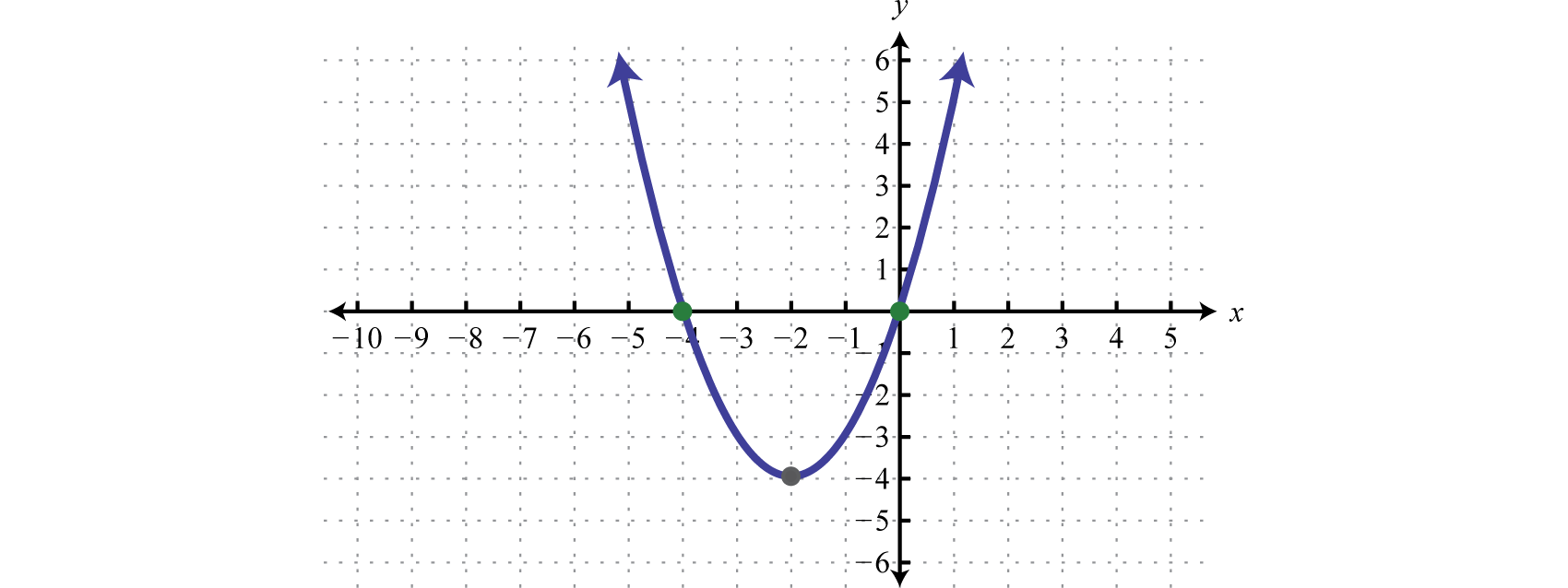### Example 9

Find the roots: $f(x)=x4−5x2+4.$

Solution:

To find roots we set the function equal to zero and solve.

$f(x)=0x4−5x2+4=0(x2−1)(x2−4)=0(x+1)(x−1)(x+2)(x−2)=0$

Next, set each factor equal to zero and solve.

$x+1=0 or x−1=0 or x+2=0 or x−2=0x=−1x=1x=−2x=2$

Answer: The roots are −1, 1, −2, and 2.

Graphing the previous function is not within the scope of this course. However, the graph is provided below: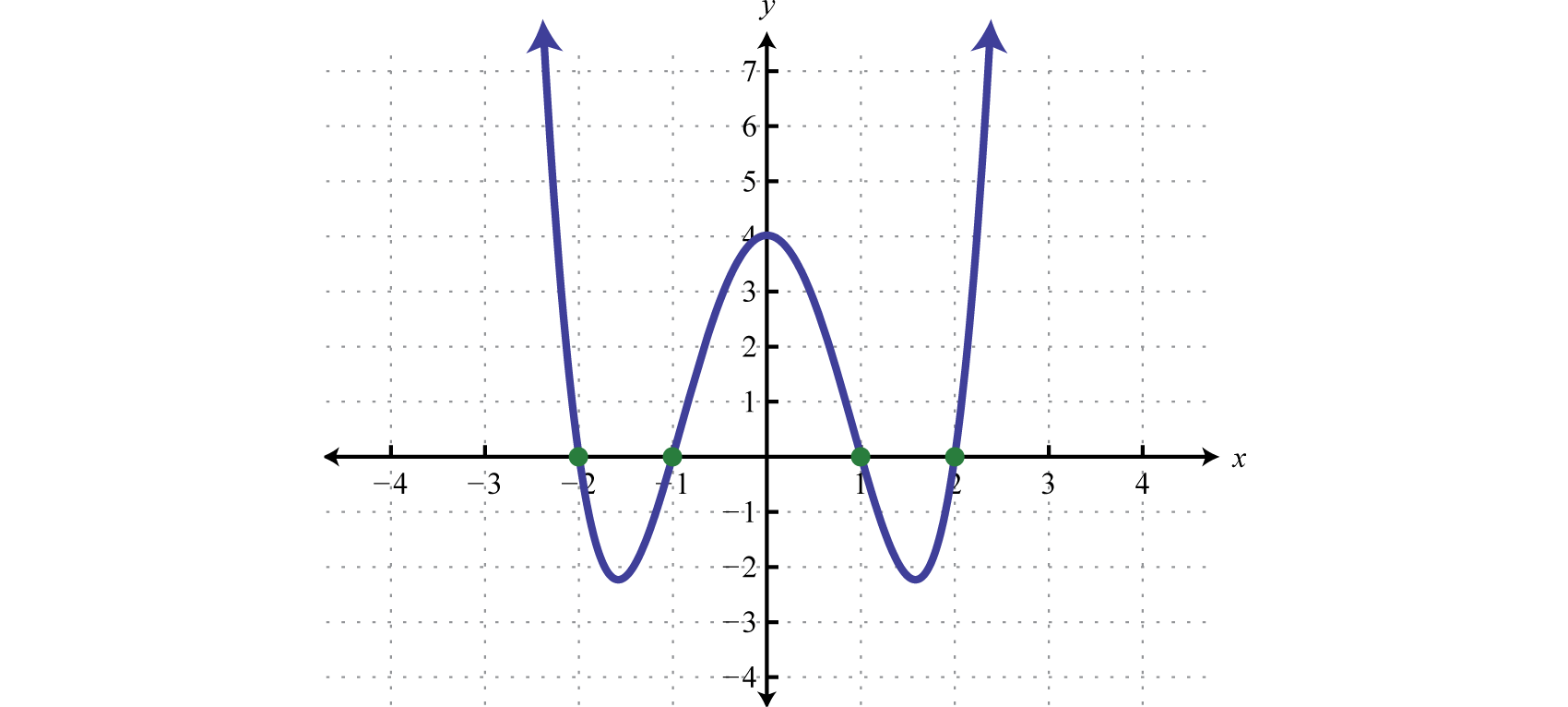Notice that the degree of the polynomial is 4 and we obtained four roots. In general, for any polynomial function with one variable of degree n, the fundamental theorem of algebraGuarantees that there will be as many (or fewer) roots to a polynomial function with one variable as its degree. guarantees n real roots or fewer. We have seen that many polynomials do not factor. This does not imply that functions involving these unfactorable polynomials do not have real roots. In fact, many polynomial functions that do not factor do have real solutions. We will learn how to find these types of roots as we continue in our study of algebra.

### Example 10

Find the roots: $f(x)=−x2+10x−25.$

Solution:

To find roots we set the function equal to zero and solve.

$f(x)=0−x2+10x−25=0−(x2−10x+25)=0−(x−5)(x−5)=0$

Next, set each variable factor equal to zero and solve.

$x−5=0orx−5=0=5x=5$

A solution that is repeated twice is called a double rootA root that is repeated twice.. In this case, there is only one solution.

The previous example shows that a function of degree 2 can have one root. From the factoring step, we see that the function can be written

$f(x)=−(x−5)2$

In this form, we can see a reflection about the x-axis and a shift to the right 5 units. The vertex is the x-intercept, illustrating the fact that there is only one root.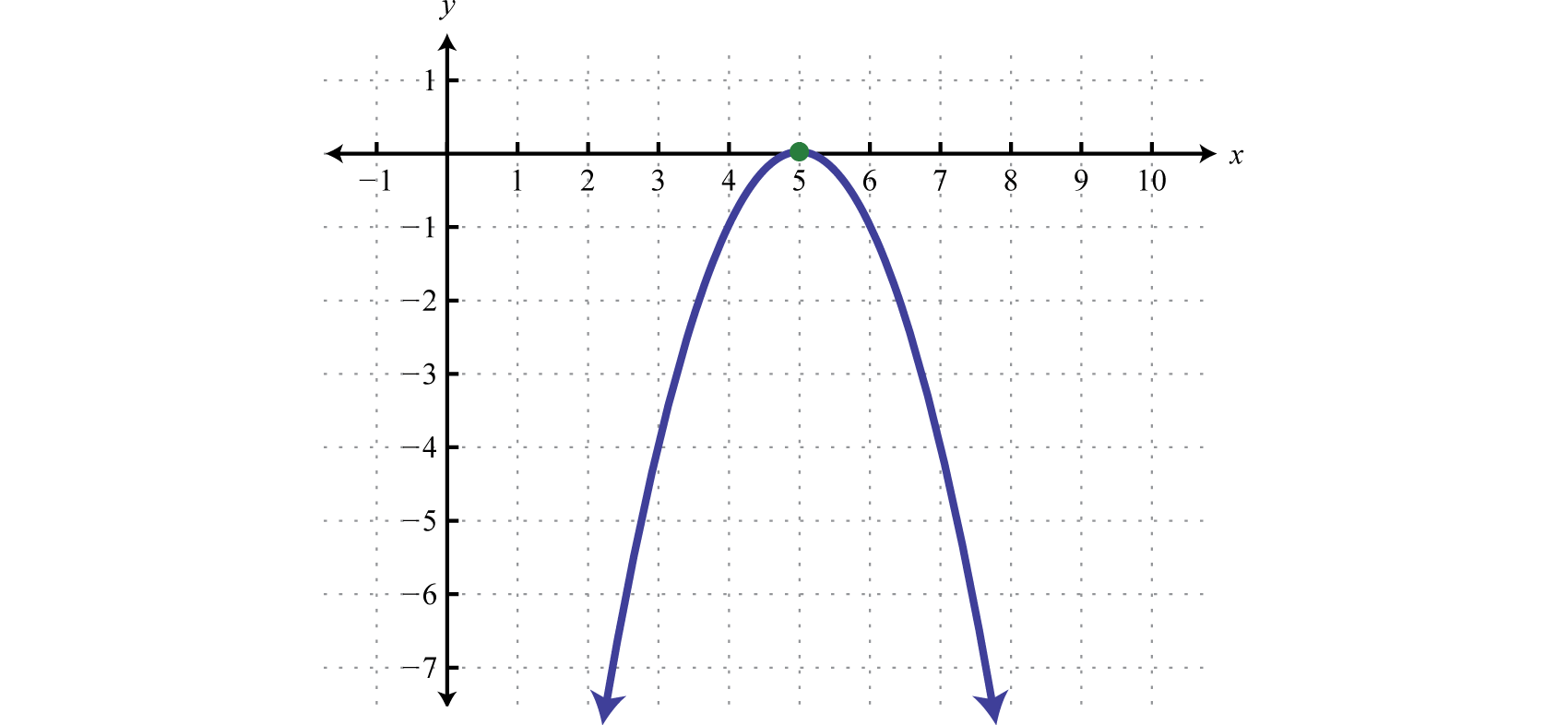Try this! Find the roots of $f(x)=x3+3x2−x−3.$

### Example 11

Assuming dry road conditions and average reaction times, the safe stopping distance in feet is given by $d(x)=120x2+x$, where x represents the speed of the car in miles per hour. Determine the safe speed of the car if you expect to stop in 40 feet.

Solution:

We are asked to find the speed x where the safe stopping distance $d(x)=40$ feet.

$d(x)=40120x2+x=40$

To solve for x, rewrite the resulting equation in standard form. In this case, we will first multiply both sides by 20 to clear the fraction.

$20(120x2+x)=20(40)x2+20x=800x2+20x−800=0$

Next factor and then set each factor equal to zero.

$x2+20x−800=0(x+40)(x−20)=0x+40=0orx−20=0x=−40x=20$

The negative answer does not make sense in the context of this problem. Consider $x=20$ miles per hour to be the only solution.

## Finding Equations with Given Solutions

We can use the zero-product property to find equations, given the solutions. To do this, the steps for solving by factoring are performed in reverse.

### Example 12

Find a quadratic equation with integer coefficients, given solutions $−32$ and $13.$

Solution:

Given the solutions, we can determine two linear factors. To avoid fractional coefficients, we first clear the fractions by multiplying both sides by the denominator.

$x=−32orx=132x=−33x=12x+3=03x−1=0$

The product of these linear factors is equal to zero when $x=−32$ or $x=13.$

$(2x+3)(3x−1)=0$

Multiply the binomials and present the equation in standard form.

$6x2−2x+9x−3=06x2+7x−3=0$

We may check our equation by substituting the given answers to see if we obtain a true statement. Also, the equation found above is not unique and so the check becomes essential when our equation looks different from someone else’s. This is left as an exercise.

Answer: $6x2+7x−3=0$

### Example 13

Find a polynomial function with real roots 1, −2, and 2.

Solution:

Given solutions to $f(x)=0$ we can find linear factors.

$x=1orx=−2orx=2x−1=0x+2=0x−2=0$

Apply the zero-product property and multiply.

$(x−1)(x+2)(x−2)=0(x−1)(x2−4)=0x3−4x−x2+4=0x3−x2−4x+4=0$

Answer: $f(x)=x3−x2−4x+4$

Try this! Find a polynomial equation with integer coefficients, given solutions $12$ and $−34.$

Answer: $8x2+2x−3=0$

### Key Takeaways

• Factoring and the zero-product property allow us to solve equations.
• To solve a polynomial equation, first write it in standard form. Once it is equal to zero, factor it and then set each variable factor equal to zero. The solutions to the resulting equations are the solutions to the original.
• Not all polynomial equations can be solved by factoring. We will learn how to solve polynomial equations that do not factor later in the course.
• A polynomial function can have at most a number of real roots equal to its degree. To find roots of a function, set it equal to zero and solve.
• To find a polynomial equation with given solutions, perform the process of solving by factoring in reverse.

### Part A: General Factoring

Factor completely.

1. $50x2−18$

2. $12x3−3x$

3. $10x3+65x2−35x$

4. $15x4+7x3−4x2$

5. $6a4b−15a3b2−9a2b3$

6. $8a3b−44a2b2+20ab3$

7. $36x4−72x3−4x2+8x$

8. $20x4+60x3−5x2−15x$

9. $3x5+2x4−12x3−8x2$

10. $10x5−4x4−90x3+36x2$

11. $x4−23x2−50$

12. $2x4−31x2−16$

13. $−2x5−6x3+8x$

14. $−36x5+69x3+27x$

15. $54x5−78x3+24x$

16. $4x6−65x4+16x2$

17. $x6−7x3−8$

18. $x6−25x3−54$

19. $3x6+4x3+1$

20. $27x6−28x3+1$

### Part B: Solving Polynomial Equations by Factoring

Solve.

1. $(6x−5)(x+7)=0$

2. $(x+9)(3x−8)=0$

3. $5x(2x−5)(3x+1)=0$

4. $4x(5x−1)(2x+3)=0$

5. $(x−1)(2x+1)(3x−5)=0$

6. $(x+6)(5x−2)(2x+9)=0$

7. $(x+4)(x−2)=16$

8. $(x+1)(x−7)=9$

9. $(6x+1)(x+1)=6$

10. $(2x−1)(x−4)=39$

11. $x2−15x+50=0$

12. $x2+10x−24=0$

13. $3x2+2x−5=0$

14. $2x2+9x+7=0$

15. $110x2−715x−16=0$

16. $14−49x2=0$

17. $6x2−5x−2=30x+4$

18. $6x2−9x+15=20x−13$

19. $5x2−23x+12=4(5x−3)$

20. $4x2+5x−5=15(3−2x)$

21. $(x+6)(x−10)=4(x−18)$

22. $(x+4)(x−6)=2(x+4)$

23. $4x3−14x2−30x=0$

24. $9x3+48x2−36x=0$

25. $13x3−34x=0$

26. $12x3−150x=0$

27. $−10x3−28x2+48x=0$

28. $−2x3+15x2+50x=0$

29. $2x3−x2−72x+36=0$

30. $4x3−32x2−9x+72=0$

31. $45x3−9x2−5x+1=0$

32. $x3−3x2−x+3=0$

33. $x4−5x2+4=0$

34. $4x4−37x2+9=0$

Find the roots of the given functions.

1. $f(x)=x2+10x−24$

2. $f(x)=x2−14x+48$

3. $f(x)=−2x2+7x+4$

4. $f(x)=−3x2+14x+5$

5. $f(x)=16x2−40x+25$

6. $f(x)=9x2−12x+4$

7. $g(x)=8x2+3x$

8. $g(x)=5x2−30x$

9. $p(x)=64x2−1$

10. $q(x)=4x2−121$

11. $f(x)=15x3−1x2−120x+14$

12. $f(x)=13x3+12x2−43x−2$

13. $g(x)=x4−13x2+36$

14. $g(x)=4x4−13x2+9$

15. $f(x)=(x+5)2−1$

16. $g(x)=−(x+5)2+9$

17. $f(x)=−(3x−5)2$

18. $g(x)=−(x+2)2+4$

Given the graph of a function, determine the real roots.

1.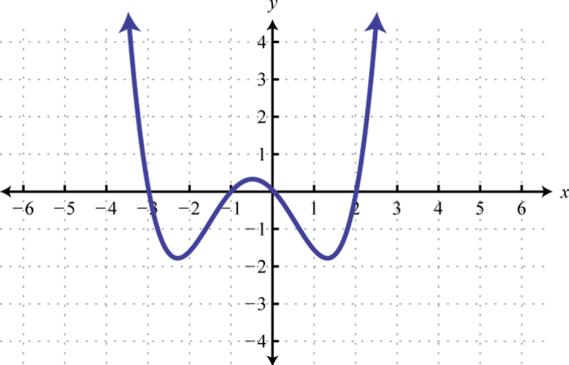2.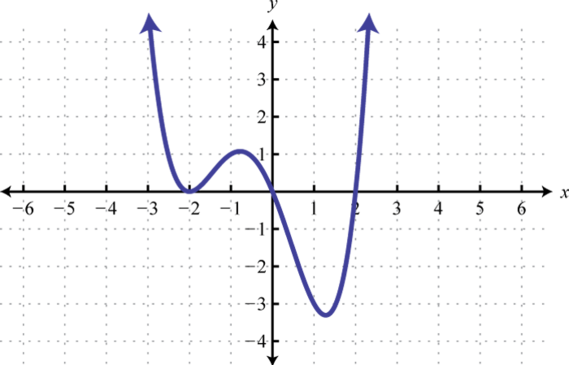3.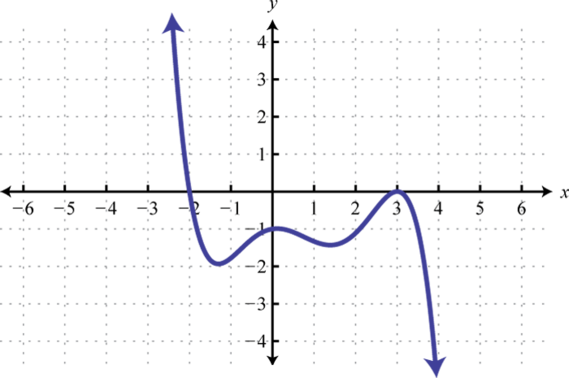4.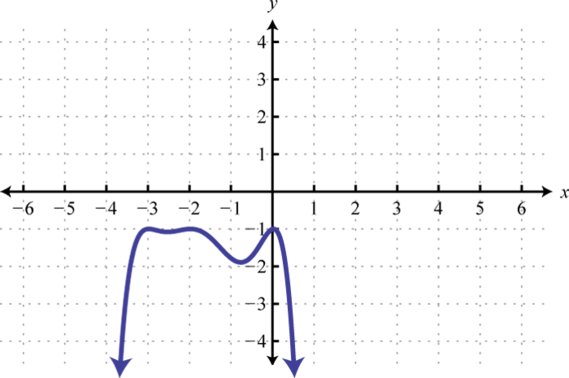5. The sides of a square measure $x−2$ units. If the area is 36 square units, then find x.

6. The sides of a right triangle have lengths that are consecutive even integers. Find the lengths of each side. (Hint: Apply the Pythagorean theorem)

7. The profit in dollars generated by producing and selling n bicycles per week is given by the formula $P(n)=−5n2+400n−6000.$ How many bicycles must be produced and sold to break even?

8. The height in feet of an object dropped from the top of a 64-foot building is given by $h(t)=−16t2+64$ where t represents the time in seconds after it is dropped. How long will it take to hit the ground?

9. A box can be made by cutting out the corners and folding up the edges of a square sheet of cardboard. A template for a cardboard box of height 2 inches is given.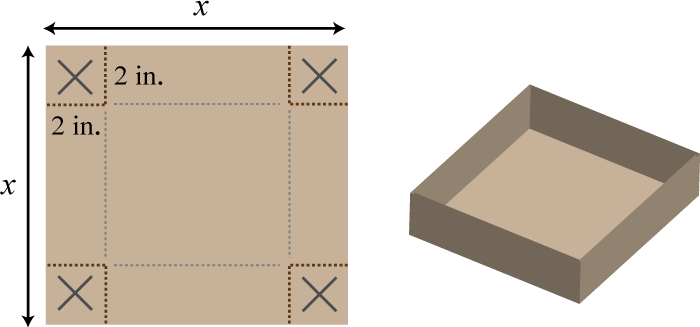What is the length of each side of the cardboard sheet if the volume of the box is to be 98 cubic inches?

10. The height of a triangle is 4 centimeters less than twice the length of its base. If the total area of the triangle is 48 square centimeters, then find the lengths of the base and height.

11. A uniform border is to be placed around an $8×10$ inch picture.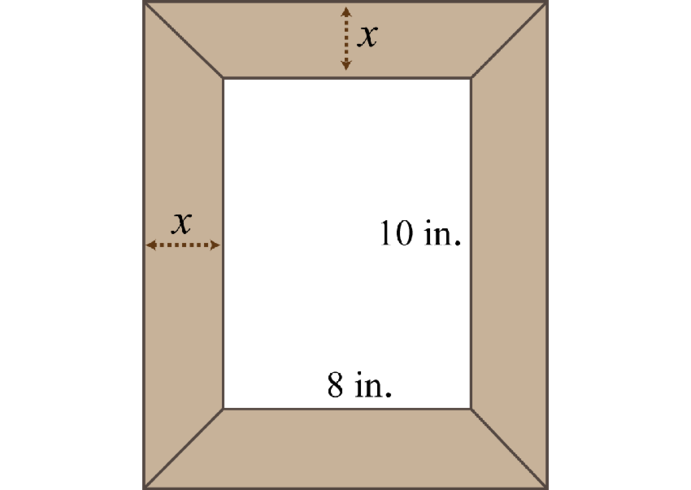If the total area including the border must be 168 square inches, then how wide should the border be?

12. The area of a picture frame including a 3-inch wide border is 120 square inches.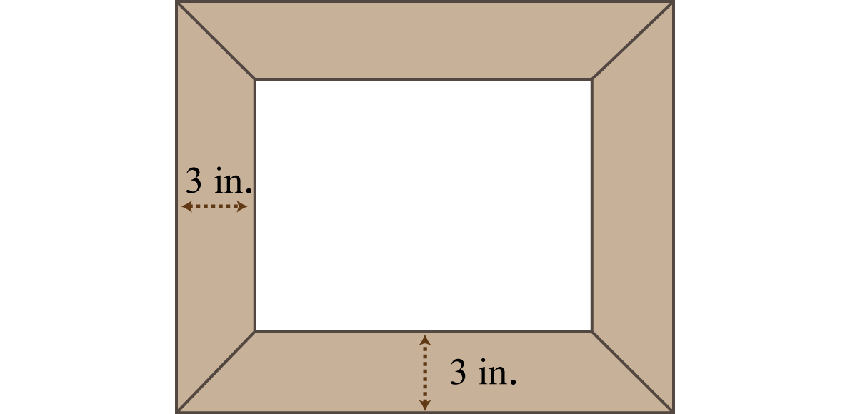If the width of the inner area is 2 inches less than its length, then find the dimensions of the inner area.

13. Assuming dry road conditions and average reaction times, the safe stopping distance in feet is given by $d(x)=120x2+x$ where x represents the speed of the car in miles per hour. Determine the safe speed of the car if you expect to stop in 75 feet.

14. A manufacturing company has determined that the daily revenue in thousands of dollars is given by the formula $R(n)=12n−0.6n2$ where n represents the number of palettes of product sold $(0≤n<20)$. Determine the number of palettes sold in a day if the revenue was 45 thousand dollars.

### Part C: Finding Equations with Given Solutions

Find a polynomial equation with the given solutions.

1. −3, 5

2. −1, 8

3. 2, $13$

4. $−34$, 5

5. 0, −4

6. 0,7

7. ±7

8. ±2

9. −3, 1, 3

10. −5, −1, 1

Find a function with the given roots.

1. $12$, $23$

2. $25$, $−13$

3. $±34$

4. $±52$

5. 5 double root

6. −3 double root

7. −1, 0, 3

8. −5, 0, 2

Recall that if $|X|=p$, then $X=−p$ or $X=p.$ Use this to solve the following absolute value equations.

1. $|x2−8|=8$

2. $|2x2−9|=9$

3. $|x2−2x−1|=2$

4. $|x2−8x+14|=2$

5. $|2x2−4x−7|=9$

6. $|x2−3x−9|=9$

### Part D: Discussion Board

1. Explain to a beginning algebra student the difference between an equation and an expression.

2. What is the difference between a root and an x-intercept? Explain.

3. Create a function with three real roots of your choosing. Graph it with a graphing utility and verify your results. Share your function on the discussion board.

4. Research and discuss the fundamental theorem of algebra.

1. $2(5x+3)(5x−3)$

2. $5x(x+7)(2x−1)$

3. $3a2b(2a+b)(a−3b)$

4. $4x(x−2)(3x+1)(3x−1)$

5. $x2(3x+2)(x+2)(x−2)$

6. $(x2+2)(x+5)(x−5)$

7. $−2x(x2+4)(x−1)(x+1)$
8. $6x(x+1)(x−1)(3x+2)(3x−2)$

9. $(x+1)(x2−x+1)(x−2)(x2+2x+4)$

10. $(3x3+1)(x+1)(x2−x+1)$

1. −7, $56$

2. 0, $52$, $−13$

3. $−12$, 1, $53$

4. −6, 4

5. $−53$, $12$

6. 5, 10

7. $−53$, 1

8. $−13$, 5

9. $−16$, 6

10. $35$, 8

11. 2, 6

12. 0, $−32$, 5

13. 0, $±32$

14. −4, 0, $65$

15. ±6, $12$

16. $±13$, $15$

17. ±1, ±2

18. 2, −12

19. $−12$, 4

20. $54$

21. $−38$, 0

22. $±18$

23. $±12$, 5

24. ±2, ±3

25. −6, −4

26. $53$

27. −3, −1, 0, 2

28. −2, 3

29. 8 units

30. 20 or 60 bicycles

31. 11 in

32. 2 inches

33. 30 miles per hour

1. $x2−2x−15=0$

2. $3x2−7x+2=0$

3. $x2+4x=0$

4. $x2−49=0$

5. $x3−x2−9x+9=0$

6. $f(x)=6x2−7x+2$

7. $f(x)=16x2−9$

8. $f(x)=x2−10x+25$

9. $f(x)=x3−2x2−3x$

10. ±4, 0

11. ±1, 3

12. −2, 1, 4

## 4.5 Rational Functions: Multiplication and Division

### Learning Objectives

1. Identify restrictions to the domain of a rational function.
2. Simplify rational functions.
3. Multiply and divide rational functions.

## Identifying Restrictions and Simplifying Rational Functions

Rational functionsFunctions of the form $r(x)=p(x)q(x)$, where $p(x)$ and $q(x)$ are polynomials and $q(x)≠0.$ have the form $r(x)=p(x)q(x),$ where $p(x)$ and $q(x)$ are polynomials and $q(x)≠0.$ The domain of a rational functionThe set of real numbers for which the rational function is defined. consists of all real numbers x except those where the denominator $q(x)=0.$ RestrictionsThe set of real numbers for which a rational function is not defined. are the real numbers for which the expression is not defined. We often express the domain of a rational function in terms of its restrictions. For example, consider the function

$f(x)=x2−4x+3x2−5x+6$

which can be written in factored form

$f(x)=(x−1)(x−3)(x−2)(x−3)$

Because rational expressions are undefined when the denominator is 0, we wish to find the values for x that make it 0. To do this, apply the zero-product property. Set each factor in the denominator equal to 0 and solve.

$(x−2)(x−3)=0x−2=0 or x−3=0 x=2 x=3$

Therefore, the original function is defined for any real number except 2 and 3. We can express its domain using notation as follows:

$Set-builder notation Interval notation {x|x≠2,3} (−∞,2)∪(2,3)∪(3,∞)$

The restrictions to the domain of a rational function are determined by the denominator. Once the restrictions are determined we can cancel factors and obtain an equivalent function as follows:It is important to note that 1 is not a restriction to the domain because the expression is defined as 0 when the numerator is 0. In fact, $x=1$ is a root. This function is graphed below: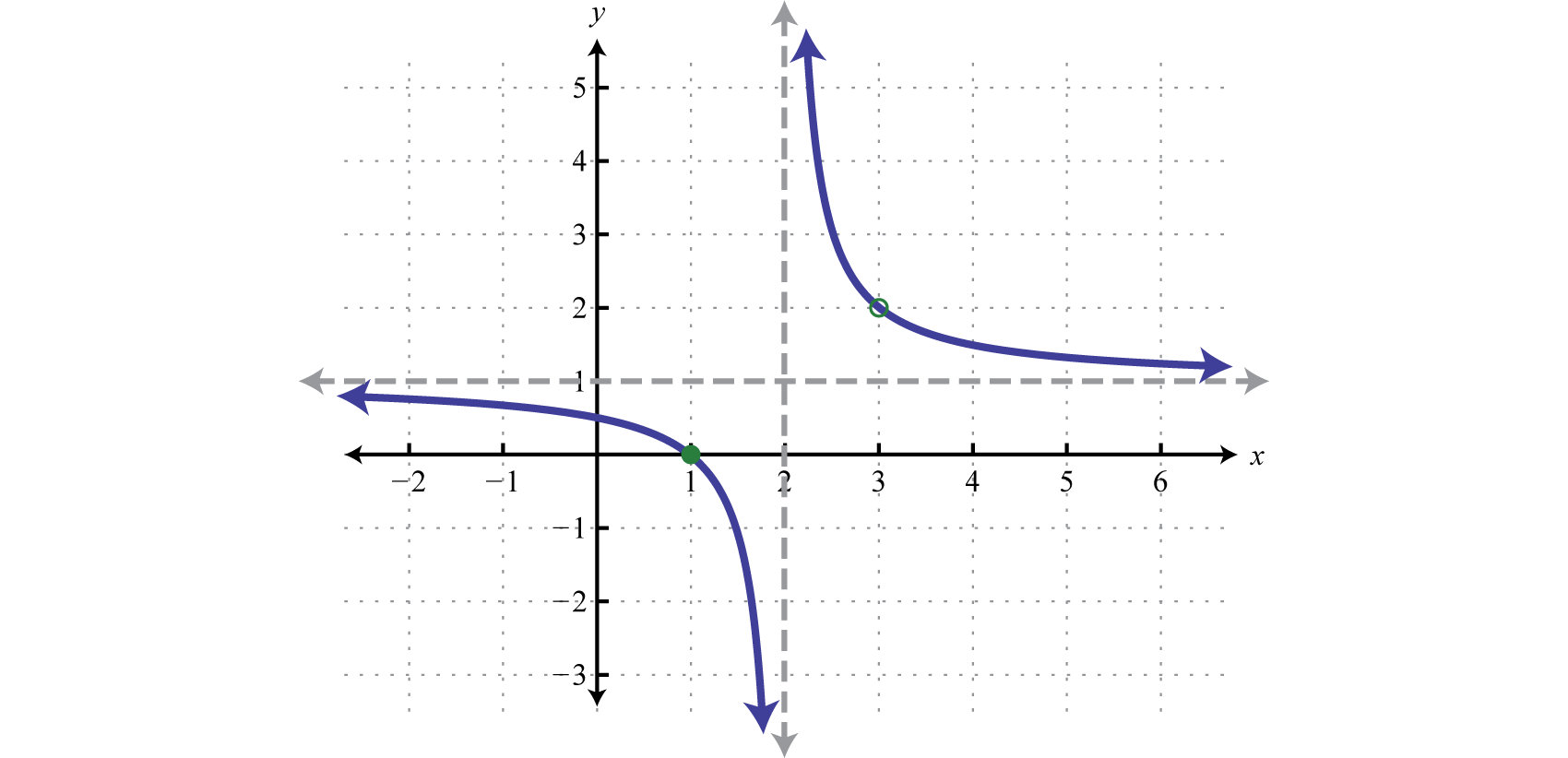Notice that there is a vertical asymptote at the restriction $x=2$ and the graph is left undefined at the restriction $x=3$ as indicated by the open dot, or hole, in the graph. Graphing rational functions in general is beyond the scope of this textbook. However, it is useful at this point to know that the restrictions are an important part of the graph of rational functions.

### Example 1

State the restrictions and simplify: $g(x)=24x76x5.$

Solution:

In this example, the function is undefined where x is 0.

$g(0)=24(0)76(0)5=00 undefined$

Therefore, the domain consists of all real numbers x, where $x≠0.$ With this understanding, we can simplify by reducing the rational expression to lowest terms. Cancel common factors.

$g(x)=244x7x26x5=4x2$

Answer: $g(x)=4x2$, where $x≠0$

### Example 2

State the restrictions and simplify: $f(x)=2x2+5x−34x2−1.$

Solution:

First, factor the numerator and denominator.

$f(x)=2x2+5x−34x2−1=(2x−1)(x+3)(2x+1)(2x−1)$

Any x-value that makes the denominator zero is a restriction. To find the restrictions, first set the denominator equal to zero and then solve

$(2x+1)(2x−1)=02x+1=0 or 2x−1=0 2x=−1 2x=1 x=−12 x=12$

Therefore, $x≠±12.$ With this understanding, we can cancel any common factors.

$f(x)=(2x−1)(x+3)(2x+1)(2x−1)=x+32x+1$

Answer: $f(x)=x+32x+1$, where $x≠±12$

We define the opposite of a polynomial P to be −P. Finding the opposite of a polynomial requires the application of the distributive property. For example, the opposite of the polynomial $(x−3)$ is written as

$−(x−3)=−1⋅(x−3)=−x+3=3−x$

This leads us to the opposite binomial propertyIf given a binomial $a−b$, then the opposite is $−(a−b)=b−a.$, $−(a−b)=(b−a).$ Care should be taken not to confuse this with the fact that $(a+b)=(b+a).$ This is the case because addition is commutative. In general,

$−(a−b)=(b−a)orb−aa−b=−1(a+b)=(b+a)orb+aa+b=1$

Also, it is important to recall that

$−ab=−ab=a−b$

In other words, a negative fraction is shown by placing the negative sign in either the numerator, in front of the fraction bar, or in the denominator. Generally, negative denominators are avoided.

### Example 3

State the restrictions and simplify: $25−x2x2−10x+25.$

Solution:

Begin by factoring the numerator and denominator.

$25−x2x2−10x+25=(5−x)(5+x)(x−5)(x−5)=−1⋅(x−5)(5+x)(x−5)(x−5)Opposite binomial property =−1⋅(x−5)(5+x)(x−5)(x−5)Cancel.=−x+5x−5$

Answer: $−x+5x−5$, where $x≠5$

It is important to remember that we can only cancel factors of a product. A common mistake is to cancel terms. For example,

$x2+7x−30x2−7x+12 incorrect! x+10x−4incorrect! 2x−1x−1incorrect!$

Try this! State the restrictions and simplify: $x−2x24x4−x2.$

Answer: $−1x(2x+1)$, where $x≠0,±12$

In some examples, we will make a broad assumption that the denominator is nonzero. When we make that assumption, we do not need to determine the restrictions.

### Example 4

Simplify: $x3−2x2y+4xy2−8y3x4−16y4.$ (Assume all denominators are nonzero.)

Solution:

Factor the numerator by grouping. Factor the denominator using the formula for a difference of squares.

$x3+4xy2−2x2y−8y3x4−16y4=x(x2+4y2)−2y(x2+4y2)(x2+4y2)(x2−4y2)=(x2+4y2)(x−2y)(x2+4y2)(x+2y)(x−2y)$

Next, cancel common factors.

$=(x2+4y2)1(x−2y)1(x2+4y2)(x+2y)(x−2y)=1x+2y$

Note: When the entire numerator or denominator cancels out a factor of 1 always remains.

Answer: $1x+2y$

### Example 5

Given $f(x)=x2−2x+5$, simplify $f(x)−f(3)x−3.$

Solution:

Begin by calculating $f(3).$

$f(3)=(3)2−2(3)+5=9−6+5=3+5=8$

Next, substitute into the quotient that is to be simplified.

$f(x)−f(3)x−3=x2−2x+5−8x−3=x2−2x−3x−3=(x+1)(x−3)(x−3)=x+1$

Answer: $x+1$, where $x≠3$

An important quantity in higher level mathematics is the difference quotientThe mathematical quantity $f(x+h)−f(x)h$, where $h≠0$, which represents the slope of a secant line through a function f.:

$f(x+h)−f(x)h, where h≠0$

This quantity represents the slope of the line connecting two points on the graph of a function. The line passing through the two points is called a secant lineLine that intersects two points on the graph of a function..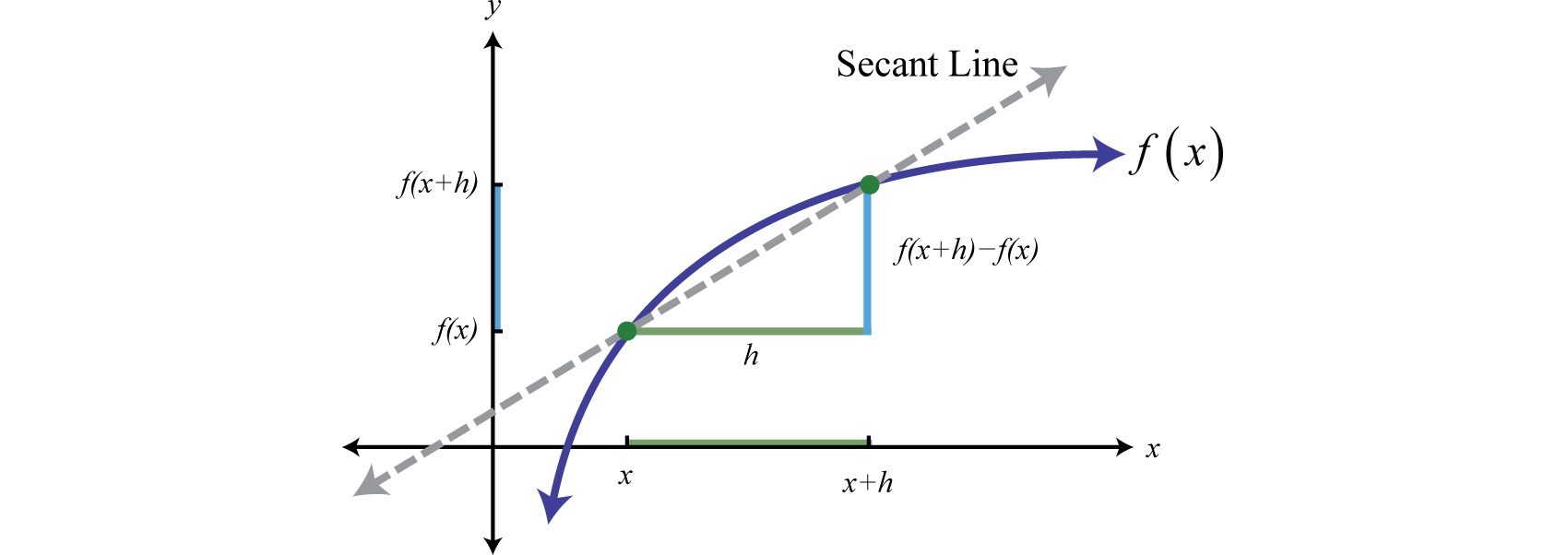Calculating the difference quotient for many different functions is an important skill to learn in intermediate algebra. We will encounter this quantity often as we proceed in this textbook. When calculating the difference quotient we assume the denominator is nonzero.

### Example 6

Given $g(x)=−2x2+1$, simplify $g(x+h)−g(x)h.$

Solution:

$g(x+h)−g(x)h=(−2(x+h)2+1)−(−2x2+1)h=−2(x2+2xh+h2)+1+2x2−1h=−2x2−4xh−2h2+1+2x2−1h=−4xh−2h2h=−4x−2h$

Answer: $−4x−2h$

Try this! Given $f(x)=x2−x−1$, simplify $f(x+h)−f(x)h.$

Answer: $2x−1+h$

## Multiplying and Dividing Rational Functions

When multiplying fractions, we can multiply the numerators and denominators together and then reduce. Multiplying rational expressions is performed in a similar manner. In general, given polynomials P, Q, R, and S, where $Q≠0$ and $S≠0$, we have

$PQ⋅RS=PRQS$

The restrictions to the domain of a product consist of the restrictions of each function.

### Example 7

Given $f(x)=9x2−25x−5$ and $g(x)=x2−2x−153x+5$, find $(f⋅g)(x)$ and determine the restrictions to the domain.

Solution:

In this case, the domain of $f$ consists of all real numbers except 5, and the domain of $g$ consists of all real numbers except $−53.$ Therefore, the domain of the product consists of all real numbers except 5 and $−53.$ Multiply the functions and then simplify the result.

$(f⋅g)(x)=f(x)⋅g(x)=9x2−25x−5⋅x2−2x−153x+5=(3x+5)(3x−5)x−5⋅(x−5)(x+3)3x+5 Factor.=(3x+5)(3x−5)(x−5)(x+3)(x−5)(3x+5) Cancel.=(3x−5)(x+3)$

Answer: $(f⋅g)(x)=(3x−5)(x+3)$, where $x≠5, −53$

To divide two fractions, we multiply by the reciprocal of the divisor. Dividing rational expressions is performed in a similar manner. In general, given polynomials P, Q, R, and S, where $Q≠0$, $R≠0$, and $S≠0$, we have

$PQ÷RS=PQ⋅SR=PSQR$

The restrictions to the domain of a quotient will consist of the restrictions of each function as well as the restrictions on the reciprocal of the divisor.

### Example 8

Given $f(x)=2x2+13x−7x2−4x−21$ and $g(x)=2x2+5x−349−x2$, find $(f/g)(x)$ and determine the restrictions.

Solution:

$(f/g)(x)= f(x) ÷ g(x)=2x2+13x−7x2−4x−21÷2x2+5x−349−x2=2x2+13x−7x2−4x−21⋅49−x22x2+5x−3Multiply by the reciprocal of the divisor.=(2x−1)(x+7)(x+3)(x−7)⋅(7+x)(7−x)(2x−1)(x+3)Factor.=(2x−1)(x+7)(7+x)(−1)(x−7)(x+3)(x−7)(2x−1)(x+3)Cancel.=−(x+7)2(x+3)2$

In this case, the domain of $f$ consists of all real numbers except −3 and 7, and the domain of $g$ consists of all real numbers except 7 and −7. In addition, the reciprocal of $g(x)$ has a restriction of −3 and $12.$ Therefore, the domain of this quotient consists of all real numbers except −3, $12$, and ±7.

Answer: $(f/g)(x)=−(x+7)2(x+3)2$, where $x≠−3,12,±7$

Recall that multiplication and division operations are to be performed from left to right.

### Example 9

Simplify: $4x2−16x2+3x÷2x+1x2+2x+1⋅27x42x2+x−1.$ (Assume all denominators are nonzero.)

Solution:

Begin by replacing the factor that is to be divided by multiplication of its reciprocal.

$4x2−16x2+3x÷2x+1x2+2x+1⋅27x42x2+x−1=4x2−16x2+3x⋅x2+2x+12x+1⋅27x42x2+x−1=(2x+1)(2x−1)3x(2x+1)⋅(x+1)(x+1)(2x+1)⋅27x4(2x−1)(x+1)=(2x+1)(2x−1)(x+1)(x+1)⋅279x4x33x(2x+1)(2x+1)(2x−1)(x+1)=9x3(x+1)(2x+1)$

Answer: $9x3(x+1)(2x+1)$

Try this! Given $f(x)=2x+53x2+14x−5$ and $g(x)=6x2+13x−5x+5$, calculate $(f/g)(x)$ and determine the restrictions.

Answer: $(f/g)(x)=1(3x−1)2$, where $x≠−5,−52,13$

If a cost function $C$ represents the cost of producing x units, then the average costThe total cost divided by the number of units produced, which can be represented by $C–(x)=C(x)x$, where $C(x)$ is a cost function. $C–$ is the cost divided by the number of units produced.

$C–(x)=C(x)x$

### Example 10

A manufacturer has determined that the cost in dollars of producing sweaters is given by $C(x)=0.01x2−3x+1200$, where x represents the number of sweaters produced daily. Determine the average cost of producing 100, 200, and 300 sweaters per day.

Solution:

Set up a function representing the average cost.

$C–(x)=C(x)x=0.01x2−3x+1200x$

Next, calculate $C–(100)$, $C–(200)$, and $C–(300).$

$C–(100)=0.01(100)2−3(100)+1200(100)=100−300+1200100=1000100=10.00C–(200)=0.01(200)2−3(200)+1200(200)=400−600+1200200=1000200=5.00C–(300)=0.01(300)2−3(300)+1200(300)=900−900+1200300=1200300=4.00$

Answer: The average cost of producing 100 sweaters per day is \$10.00 per sweater. If 200 sweaters are produced, the average cost per sweater is \$5.00. If 300 are produced, the average cost per sweater is \$4.00.

### Key Takeaways

• Simplifying rational expressions is similar to simplifying fractions. First, factor the numerator and denominator and then cancel the common factors. Rational expressions are simplified if there are no common factors other than 1 in the numerator and the denominator.
• Simplified rational functions are equivalent for values in the domain of the original function. Be sure to state the restrictions unless the problem states that the denominators are assumed to be nonzero.
• After multiplying rational expressions, factor both the numerator and denominator and then cancel common factors. Make note of the restrictions to the domain. The values that give a value of 0 in the denominator for all expressions are the restrictions.
• To divide rational expressions, multiply the numerator by the reciprocal of the divisor.
• The restrictions to the domain of a product consist of the restrictions to the domain of each factor.

### Part A: Simplifying Rational Functions

Simplify the function and state its domain using interval notation.

1. $f(x)=25x95x5$
2. $f(x)=64x816x3$
3. $f(x)=x2−64x2+16x+64$
4. $f(x)=x2+x−20x2−25$
5. $g(x)=9−4x22x2−5x+3$
6. $g(x)=x−3x29x2−6x+1$
7. $g(x)=2x2−8x−422x2+5x−3$
8. $g(x)=6x2+5x−43x2+x−4$
9. $h(x)=x3+x2−x−1x2+2x+1$
10. $h(x)=2x3−5x2−8x+202x2−9x+10$

State the restrictions and simplify the given rational expressions.

1. $66x(2x−5)18x3(2x−5)2$
2. $26x4(5x+2)320x5(5x+2)$
3. $x2+5x+6x2−5x−14$
4. $x2−8x+12x2−2x−24$
5. $1−x25x2+x−6$
6. $4−9x23x2−8x+4$
7. $4x2+15x+99−x2$
8. $6x2+13x−525−4x2$
9. $x2−5x+4x3−x2−16x+16$
10. $x4+4x2x3+3x2+4x+12$

Simplify the given rational expressions. Assume all variable expressions in the denominator are nonzero.

1. $50ab3(a+b)2200a2b3(a+b)3$
2. $36a5b7(a−b)29a3b(a−b)$
3. $a2−b2a2+2ab+b2$
4. $a2−2ab+b2a2−b2$
5. $6x2−xy6x2−7xy+y2$
6. $y−x2x3−4x2y+2xy2$
7. $x2y2−2xy3x2y2−xy3−2y4$
8. $x4y−x2y3x3y+2x2y2+xy3$
9. $x3−x2y+xy2−y3x4−y4$
10. $y4−x4x3+x2y+xy2+y3$
11. $a2−(b+c)2(a+b)2−c2$
12. $(a+b)2−c2(a+c)2−b2$
13. $x3+y3x2+2xy+y2$
14. $x3y+x2y2+xy3x3−y3$

Given the function, simplify the rational expression.

1. Given $f(x)=x2−8$, simplify $f(x)−f(5)x−5.$

2. Given $f(x)=x2+4x−1$, simplify $f(x)−f(2)x−2.$

3. Given $g(x)=x2−3x+1$, simplify $g(x)−g(−1)x+1.$

4. Given $g(x)=x2−2x$, simplify $g(x)−g(−4)x+4.$

5. Given $f(x)=4x2+6x+1$, simplify $f(x)−f(12)2x−1.$

6. Given $f(x)=9x2+1$, simplify $f(x)−f(−13)3x+1.$

For the given function, simplify the difference quotient $f(x+h)−f(x)h, where h≠0.$

1. $f(x)=5x−3$

2. $f(x)=3−2x$

3. $f(x)=x2−3$

4. $f(x)=x2+8x$

5. $f(x)=x2−x+5$

6. $f(x)=4x2+3x−2$

7. $f(x)=ax2+bx+c$

8. $f(x)=ax2+bx$

9. $f(x)=x3+1$

10. $f(x)=x3−x+2$

### Part B: Multiplying and Dividing Rational Functions

Simplify the product $f⋅g$ and state its domain using interval notation.

1. $f(x)=52x4(x−2)2, g(x)=(x−2)312x5$
2. $f(x)=46(2x−1)315x6, g(x)=25x323(2x−1)$
3. $f(x)=10x3x2+4x+4, g(x)=x2−450x4$
4. $f(x)=25−x246x5, g(x)=12x3x2+10x+25$
5. $f(x)=5−3xx2−10x+25, g(x)=x2−6x+53x2−8x+5$
6. $f(x)=1−4x26x2+3x, g(x)=12x24x2−4x+1$

Simplify the quotient $f/g$ and state its domain using interval notation.

1. $f(x)=12x35(5x−1)3, g(x)=6x225(5x−1)4$
2. $f(x)=7x2(x+9)(x−8)2, g(x)=49x3(x+9)(x−8)4$
3. $f(x)=25x2−13x2−15x, g(x)=25x2+10x+1x3−5x2$
4. $f(x)=x2−x−62x2+13x+15, g(x)=x2−6x+94x2+12x+9$
5. $f(x)=x2−64x2, g(x)=2x2+19x+24$
6. $f(x)=2x2+11x−6, g(x)=36−x2$

Multiply or divide as indicated, state the restrictions, and simplify.

1. $14(x+12)25x3⋅45x42(x+12)3$
2. $27x620(x−7)3⋅(x−7)554x7$
3. $x2−6436x4⋅12x3x2+4x−32$
4. $50x5x2+6x−27⋅x2−81125x3$
5. $2x2+7x+53x2⋅15x3−30x22x2+x−10$
6. $3x2+14x−52x2+11x+5⋅4x2+4x+16x2+x−1$
7. $x2+4x−215x2+10x÷x2−6x+9x2+9x+14$
8. $x2−499x2−24x+16÷2x2−13x−76x2−5x−4$
9. $5x2+x−64x2−7x−15÷1−x24x2+9x+5$
10. $6x2−8x−84−9x2÷3x2−4x−49x2+12x+4$
11. $x2+4x−12x2−2x−15÷2x2−13x+186x2−31x+5$
12. $8x2+x−925x2−1÷2x2−x−110x2−3x−1$

Perform the operations and simplify. Assume all variable expressions in the denominator are nonzero.

1. $112ab⋅50a2(a−b)2a2−b2⋅6ba(a−b)$
2. $b2−a2(a−b)2⋅12a(a−b)36a2b⋅9ab(a−b)a+b$
3. $x3+y35xy⋅x2−y2x2−2xy+y2⋅25x2y(y+x)2$
4. $3xy2(2y+x)2⋅2x2+5xy+2y29x2⋅x3+8y36xy2+3y3$
5. $2x+5x−3⋅x2−95x4÷2x2+15x+2525x5$
6. $5x2−15x9x2−4⋅3x−220x3÷x−33x2−x−2$
7. $x2+5x−50x2+5x−14÷x2−25x2−49⋅x−2x2+3x−70$
8. $x2−x−564x2−4x−3÷2x2+11x−2125−9x2⋅4x2−12x+93x2−19x−40$
9. $20x2−8x−16x2+13x+6÷1−100x23x2−x−2⋅10x−12x2−3x+1$
10. $12x2−13x+1x2+18x+81÷(144x2−1)⋅x2+14x+4512x2−11x−1$
11. A manufacturer has determined that the cost in dollars of producing bicycles is given by $C(x)=0.5x2−x+6200$, where x represents the number of bicycles produced weekly. Determine the average cost of producing 50, 100, and 150 bicycles per week.

12. The cost in dollars of producing custom lighting fixtures is given by the function $C(x)=x2−20x+1200$, where x represents the number of fixtures produced in a week. Determine the average cost per unit if 20, 40, and 50 units are produced in a week.

13. A manufacturer has determined that the cost in dollars of producing electric scooters is given by the function $C(x)=3x(x−100)+32,000$, where x represents the number of scooters produced in a month. Determine the average cost per scooter if 50 are produced in a month.

14. The cost in dollars of producing a custom injected molded part is given by $C(n)=1,900+0.01n$, where n represents the number of parts produced. Calculate the average cost of each part if 2,500 custom parts are ordered.

15. The cost in dollars of an environmental cleanup is given by the function $C(p)=25,000p1−p$, where p represents the percentage of the area to be cleaned up $(0≤p<1)$. Use the function to determine the cost of cleaning up 50% of an affected area and the cost of cleaning up 80% of the area.

16. The value of a new car is given by the function $V(t)=16,500(t+1)−1$ where t represents the age of the car in years. Determine the value of the car when it is 6 years old.

### Part D: Discussion Board

1. Describe the restrictions to the rational expression $1x2−y2$. Explain.

2. Describe the restrictions to the rational expression $1x2+y2$. Explain.

3. Explain why $x=5$ is a restriction to $1x+5÷x−5x.$

4. Explain to a beginning algebra student why we cannot cancel x in the rational expression $x+2x.$

5. Research and discuss the importance of the difference quotient. What does it represent and in what subject does it appear?

1. $f(x)=5x4$;

Domain: $(−∞,0)∪(0,∞)$

2. $f(x)=x−8x+8$; Domain: $(−∞,−8)∪(−8,∞)$

3. $g(x)=−2x+3x−1$; Domain: $(−∞,1)∪(1,32)∪(32,∞)$

4. $g(x)=2(x−7)2x−1$; Domain: $(−∞,−3)∪(−3,12)∪(12,∞)$

5. $h(x)=x−1$; Domain: $(−∞,−1)∪(−1,∞)$

6. $113x2(2x−5)$; $x≠0,52$

7. $x+3x−7$; $x≠−2,7$

8. $−x+15x+6$; $x≠−65,1$

9. $−4x+33−x$; $x≠±3$

10. $1x+4$; $x≠1,±4$

11. $14a(a+b)$

12. $a−ba+b$

13. $xx−y$

14. $xx+y$

15. $1x+y$

16. $a−b−ca+b−c$

17. $x2−xy+y2x+y$

18. $x+5$, where $x≠5$

19. $x−4$, where $x≠−1$

20. $2(x+2)$, where $x≠12$

21. 5

22. $2x+h$

23. $2x−1+h$

24. $2ax+b+ah$

25. $3x2+3xh+h2$

1. $(f⋅g)(x)=13(x−2)3x$; Domain: $(−∞,0)∪(0,2)∪(2,∞)$

2. $(f⋅g)(x)=x−25x(x+2)$; Domain: $(−∞,−2)∪(−2,0)∪(0,∞)$

3. $(f⋅g)(x)=−1x−5$; Domain: $(−∞,1)∪(1,53)∪(53,5)∪(5,∞)$

4. $(f/g)(x)=10x(5x−1)$; Domain: $(−∞,0)∪(0,15)∪(15,∞)$

5. $(f/g)(x)=x(5x−1)2(5x+1)$; Domain: $(−∞,−15)∪(−15,0)∪(0,5)∪(5,∞)$

6. $(f/g)(x)=x−8x2(2x+3)$; Domain: $(−∞,−8)∪(−8,−32)∪(−32,0)∪(0,∞)$

7. $63xx+12$; $x≠−12,0$

8. $x−83x(x−4)$; $x≠−8,0,4$

9. $5(x+1)$; $x≠−52,0,2$

10. $(x+7)25x(x−3)$; $x≠−7,−2,0,3$

11. $−5x+6x−3$; $x≠−54,−1,1,3$

12. $(x+6)(6x−1)(x+3)(2x−9)$; $x≠−3,16,2,92,5$

13. $25a+b$

14. $5x(x2−xy+y2)x−y$
15. $5x(x+3)x+5$
16. $1x+5$
17. $−12x+3$
18. If 50 bicycles are produced, the average cost per bicycle is \$148. If 100 are produced, the average cost is \$111. If 150 bicycles are produced, the average cost is \$115.33.

19. If 50 scooters are produced, the average cost of each is \$490.

20. A 50% cleanup will cost \$25,000. An 80% cleanup will cost \$100,000.

## 4.6 Rational Functions: Addition and Subtraction

### Learning Objectives

1. Add and subtract rational functions.
2. Simplify complex rational expressions.

## Adding and Subtracting Rational Functions

Adding and subtracting rational expressions is similar to adding and subtracting fractions. Recall that if the denominators are the same, we can add or subtract the numerators and write the result over the common denominator. When working with rational expressions, the common denominator will be a polynomial. In general, given polynomials P, Q, and R, where $Q≠0$, we have the following:

$PQ±RQ=P±RQ$

The set of restrictions to the domain of a sum or difference of rational expressions consists of the restrictions to the domains of each expression.

### Example 1

Subtract: $4xx2−64−3x+8x2−64.$

Solution:

The denominators are the same. Hence we can subtract the numerators and write the result over the common denominator. Take care to distribute the negative 1.

$4xx2−64−3x+8x2−64=4x−(3x+8)x2−64 Subtract the numerators.=4x−3x−8x2−64 Simplify.=x−81(x+8)(x−8) Cancel.=1x+8 Restrictions x≠±8$

Answer: $1x+8$, where $x≠±8$

To add rational expressions with unlike denominators, first find equivalent expressions with common denominators. Do this just as you have with fractions. If the denominators of fractions are relatively prime, then the least common denominator (LCD) is their product. For example,

$1x+1y ⇒ LCD=x⋅y=xy$

Multiply each fraction by the appropriate form of 1 to obtain equivalent fractions with a common denominator.

$1x+1y=1⋅yx⋅y+1⋅xy⋅x =yxy+xxyEquivalent fractions with a common denominator=y+xxy$

In general, given polynomials P, Q, R, and S, where $Q≠0$ and $S≠0$, we have the following:

$PQ±RS=PS±QRQS$

### Example 2

Given $f(x)=5x3x+1$ and $g(x)=2x+1$, find $f+g$ and state the restrictions.

Solution:

Here the LCD is the product of the denominators $(3x+1)(x+1).$ Multiply by the appropriate factors to obtain rational expressions with a common denominator before adding.

$(f+g)(x)=f(x)+g(x)=5x3x+1+2x+1=5x(3x+1)⋅(x+1)(x+1)+2(x+1)⋅(3x+1)(3x+1)=5x(x+1)(3x+1)(x+1)+2(3x+1)(x+1)(3x+1)=5x(x+1)+2(3x+1)(3x+1)(x+1)=5x2+5x+6x+2(3x+1)(x+1)=5x2+11x+2(3x+1)(x+1)=(5x+1)(x+2)(3x+1)(x+1)$

The domain of f consists all real numbers except $−13$, and the domain of g consists of all real numbers except −1. Therefore, the domain of f + g consists of all real numbers except −1 and $−13.$

Answer: $(f+g)(x)=(5x+1)(x+2)(3x+1)(x+1)$, where $x≠−1,−13$

It is not always the case that the LCD is the product of the given denominators. Typically, the denominators are not relatively prime; thus determining the LCD requires some thought. Begin by factoring all denominators. The LCD is the product of all factors with the highest power.

### Example 3

Given $f(x)=3x3x−1$ and $g($